Скачать презентацию 2 -1 Chapter 2 Time Value of Money

f7850a958eefa827a1ba8791977e00ec.ppt

• Количество слайдов: 83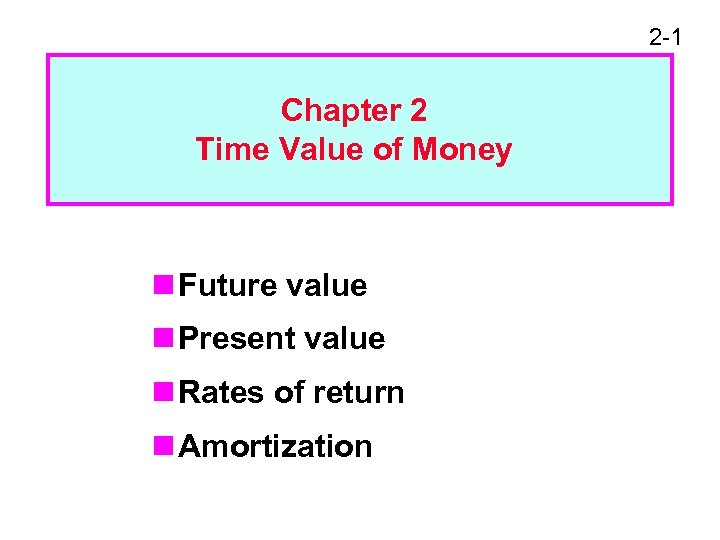2 -1 Chapter 2 Time Value of Money n Future value n Present value n Rates of return n Amortization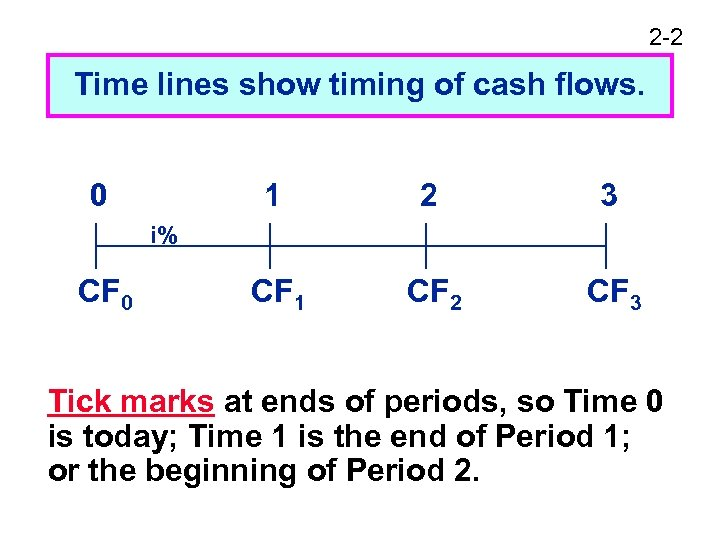2 -2 Time lines show timing of cash flows. 0 1 2 3 CF 1 CF 2 CF 3 i% CF 0 Tick marks at ends of periods, so Time 0 is today; Time 1 is the end of Period 1; or the beginning of Period 2.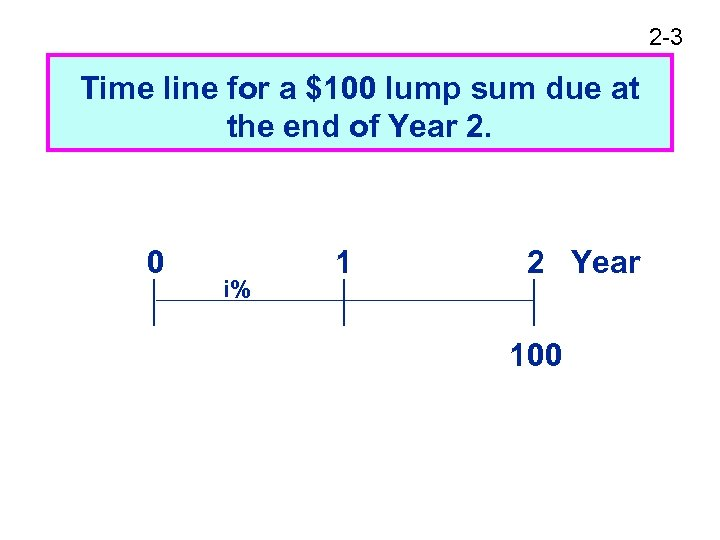2 -3 Time line for a \$100 lump sum due at the end of Year 2. 0 i% 1 2 Year 100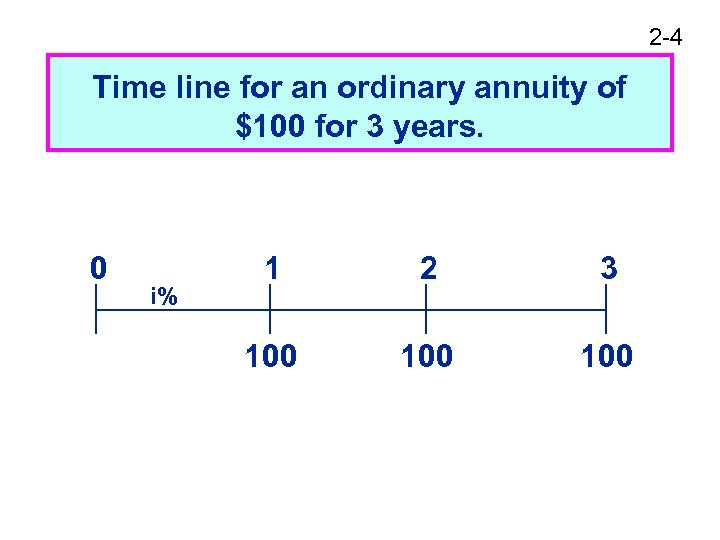2 -4 Time line for an ordinary annuity of \$100 for 3 years. 0 i% 1 2 3 100 100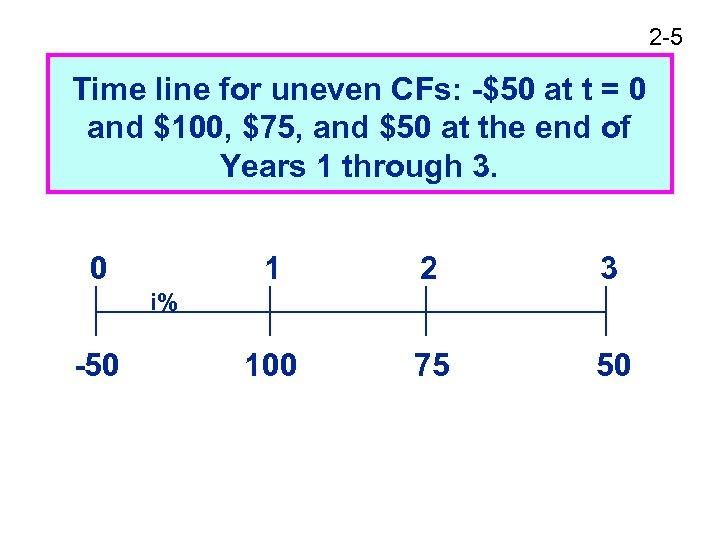2 -5 Time line for uneven CFs: -\$50 at t = 0 and \$100, \$75, and \$50 at the end of Years 1 through 3. 0 1 2 3 100 75 50 i% -50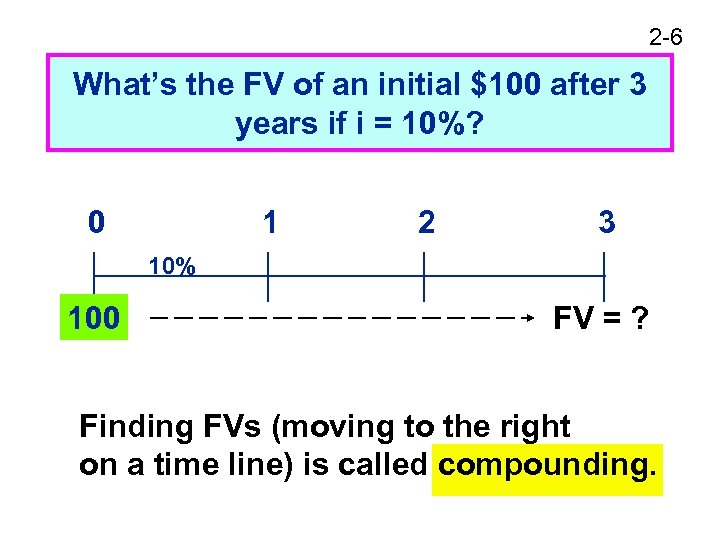2 -6 What’s the FV of an initial \$100 after 3 years if i = 10%? 0 1 2 3 10% 100 FV = ? Finding FVs (moving to the right on a time line) is called compounding.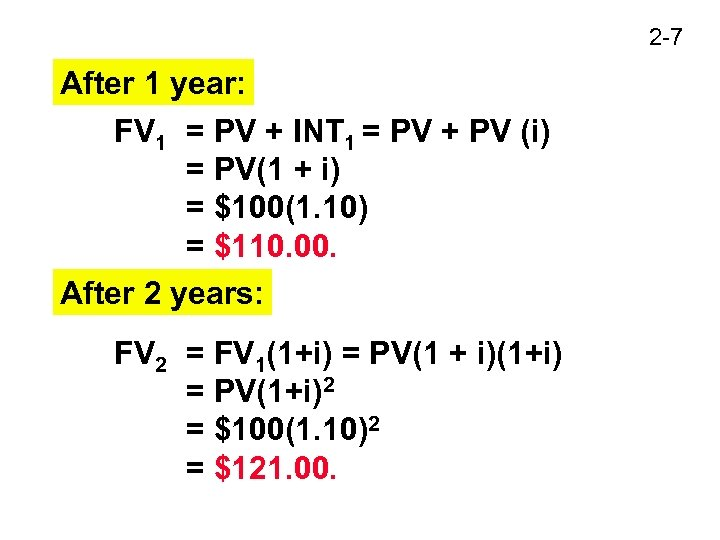2 -7 After 1 year: FV 1 = PV + INT 1 = PV + PV (i) = PV(1 + i) = \$100(1. 10) = \$110. 00. After 2 years: FV 2 = FV 1(1+i) = PV(1 + i)(1+i) = PV(1+i)2 = \$100(1. 10)2 = \$121. 00.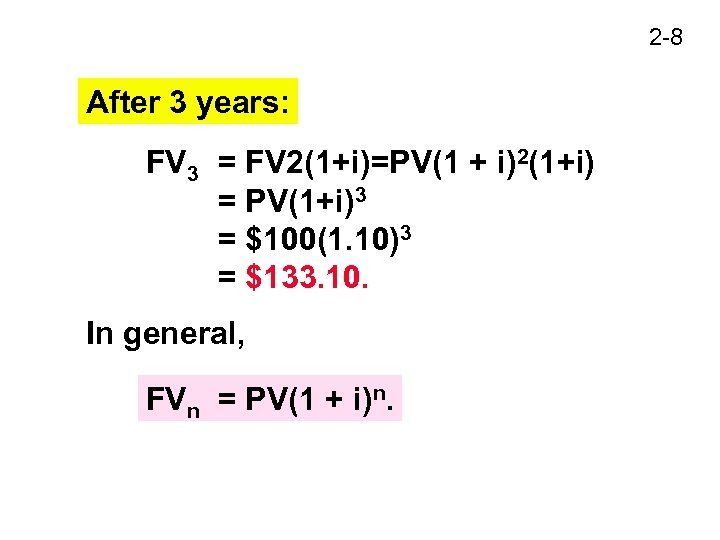2 -8 After 3 years: FV 3 = FV 2(1+i)=PV(1 + i)2(1+i) = PV(1+i)3 = \$100(1. 10)3 = \$133. 10. In general, FVn = PV(1 + i)n.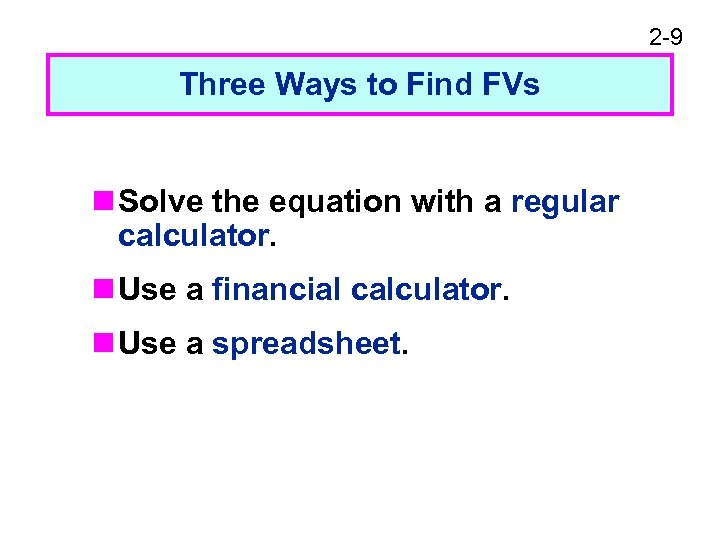2 -9 Three Ways to Find FVs n Solve the equation with a regular calculator. n Use a financial calculator. n Use a spreadsheet.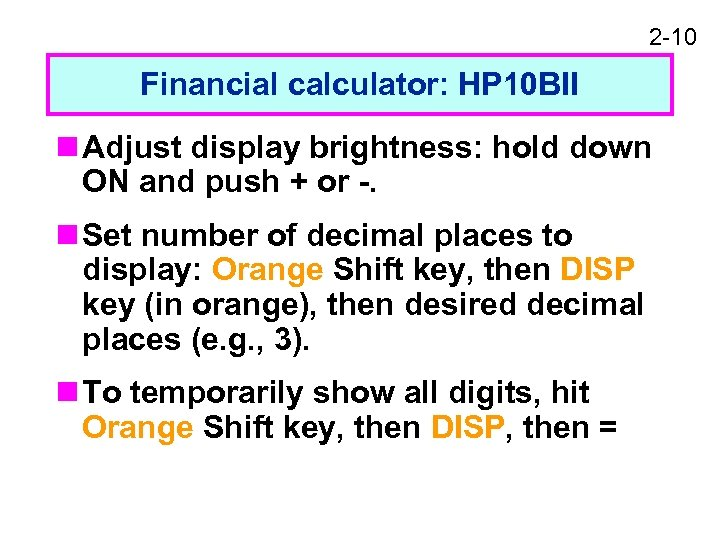2 -10 Financial calculator: HP 10 BII n Adjust display brightness: hold down ON and push + or -. n Set number of decimal places to display: Orange Shift key, then DISP key (in orange), then desired decimal places (e. g. , 3). n To temporarily show all digits, hit Orange Shift key, then DISP, then =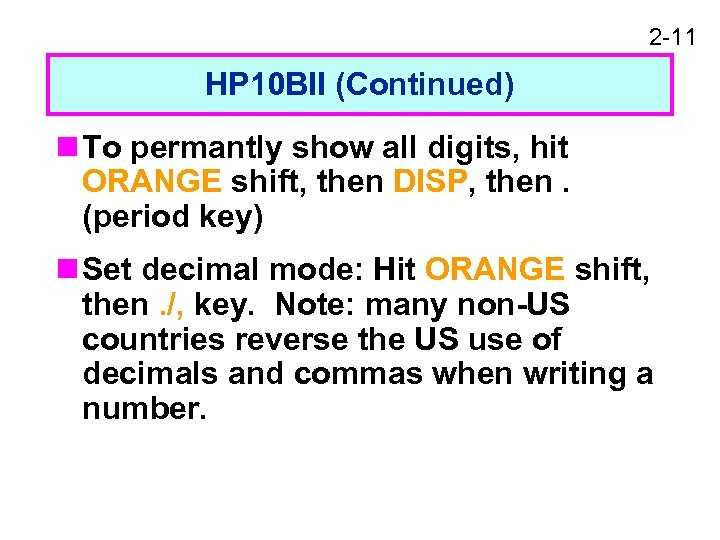2 -11 HP 10 BII (Continued) n To permantly show all digits, hit ORANGE shift, then DISP, then. (period key) n Set decimal mode: Hit ORANGE shift, then. /, key. Note: many non-US countries reverse the US use of decimals and commas when writing a number.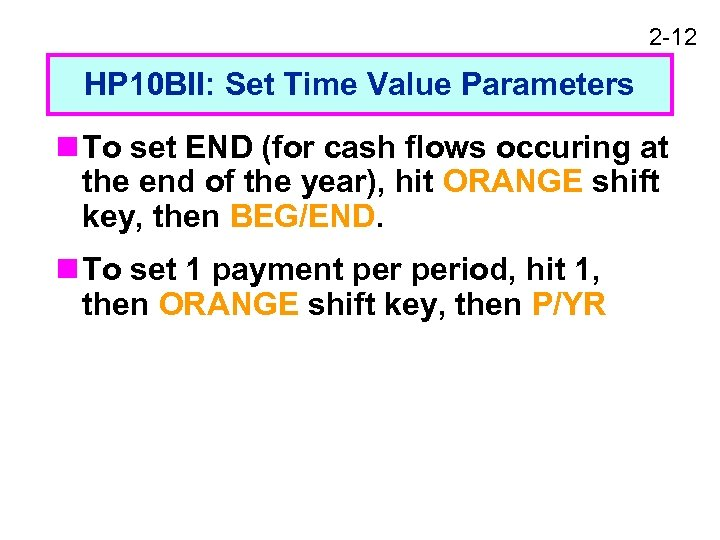2 -12 HP 10 BII: Set Time Value Parameters n To set END (for cash flows occuring at the end of the year), hit ORANGE shift key, then BEG/END. n To set 1 payment period, hit 1, then ORANGE shift key, then P/YR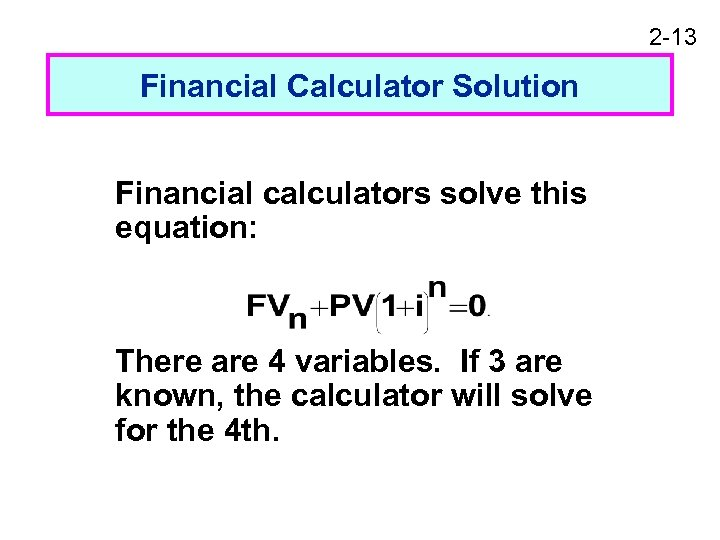2 -13 Financial Calculator Solution Financial calculators solve this equation: There are 4 variables. If 3 are known, the calculator will solve for the 4 th.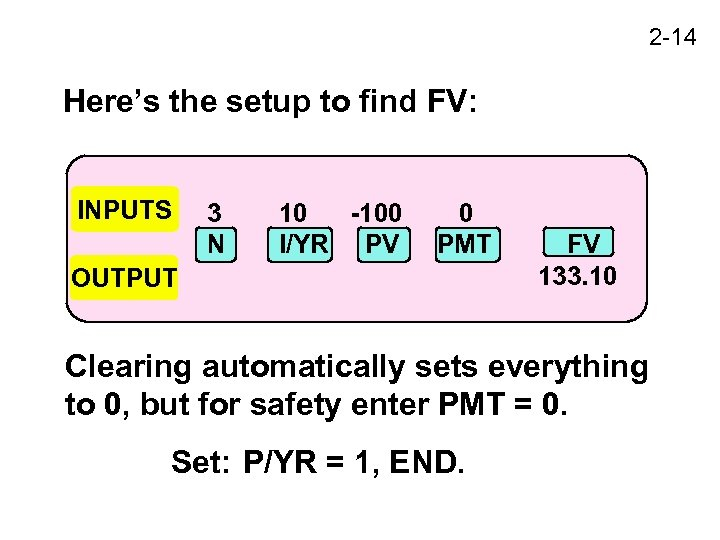2 -14 Here’s the setup to find FV: INPUTS 3 N 10 -100 I/YR PV 0 PMT OUTPUT FV 133. 10 Clearing automatically sets everything to 0, but for safety enter PMT = 0. Set: P/YR = 1, END.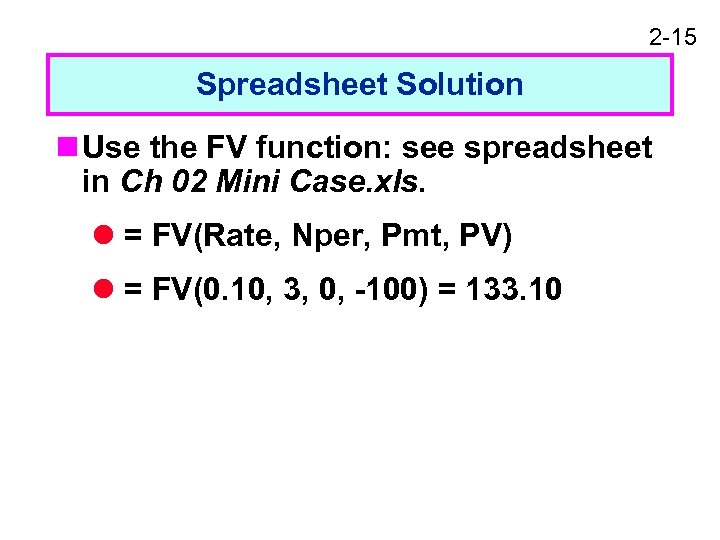2 -15 Spreadsheet Solution n Use the FV function: see spreadsheet in Ch 02 Mini Case. xls. l = FV(Rate, Nper, Pmt, PV) l = FV(0. 10, 3, 0, -100) = 133. 10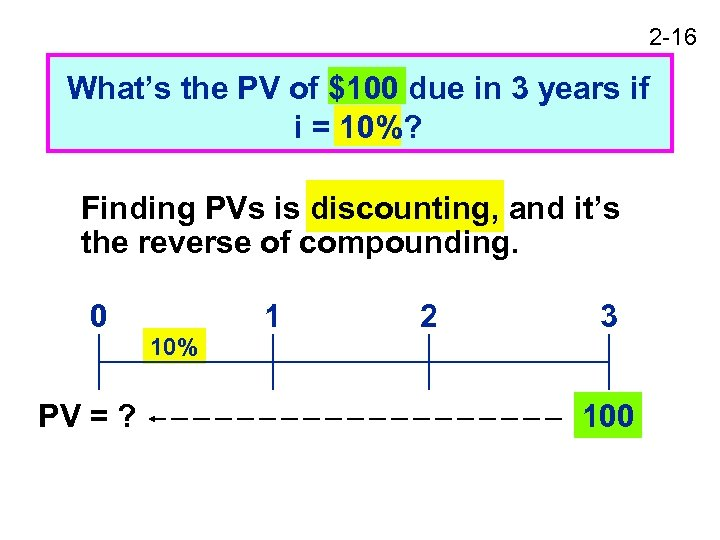2 -16 What’s the PV of \$100 due in 3 years if i = 10%? Finding PVs is discounting, and it’s the reverse of compounding. 0 PV = ? 10% 1 2 3 100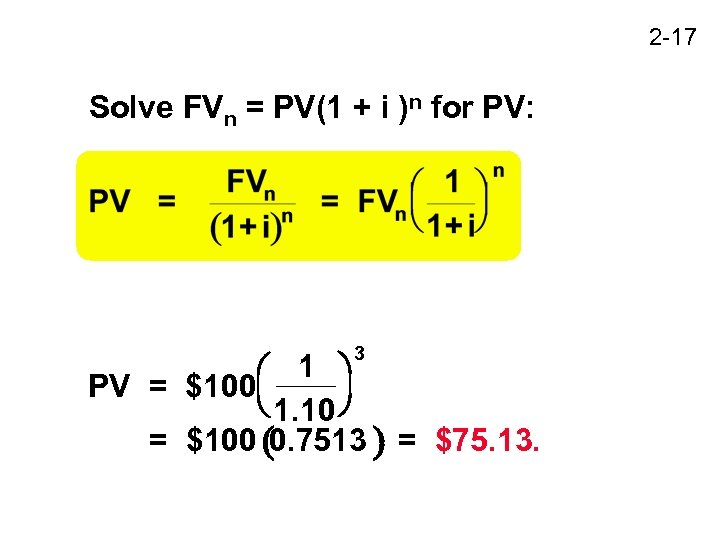2 -17 Solve FVn = PV(1 + i )n for PV: 3 1 PV = \$100 1. 10 = \$100 0. 7513 = \$75. 13.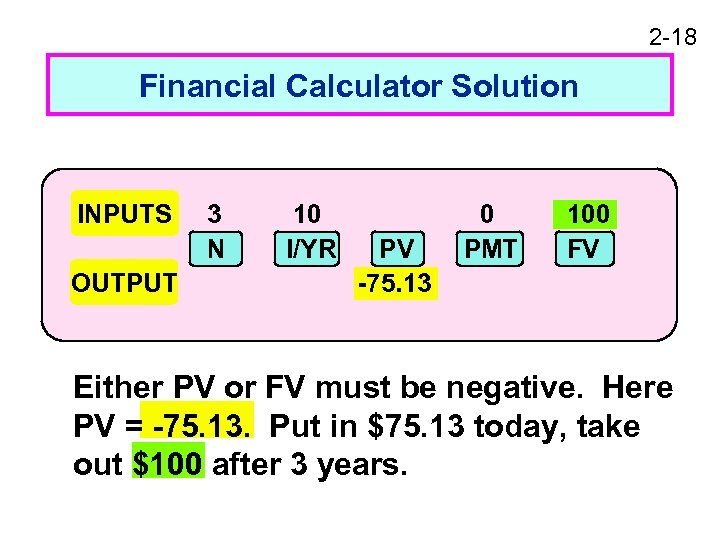2 -18 Financial Calculator Solution INPUTS OUTPUT 3 N 10 I/YR PV -75. 13 0 PMT 100 FV Either PV or FV must be negative. Here PV = -75. 13. Put in \$75. 13 today, take out \$100 after 3 years.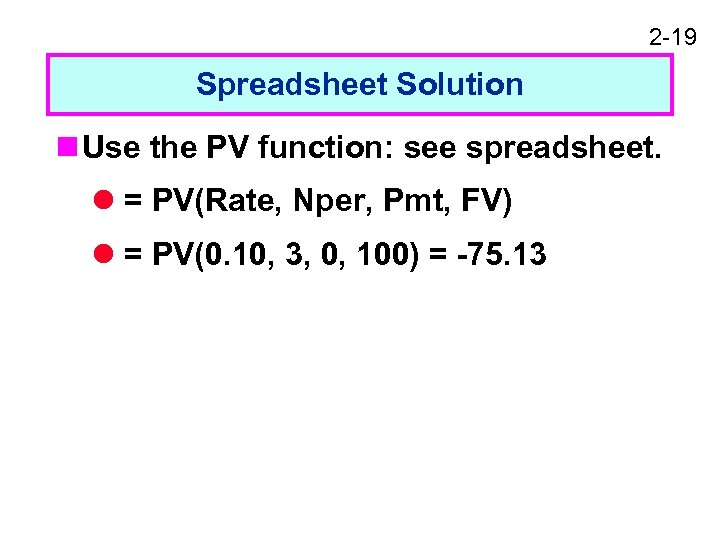2 -19 Spreadsheet Solution n Use the PV function: see spreadsheet. l = PV(Rate, Nper, Pmt, FV) l = PV(0. 10, 3, 0, 100) = -75. 13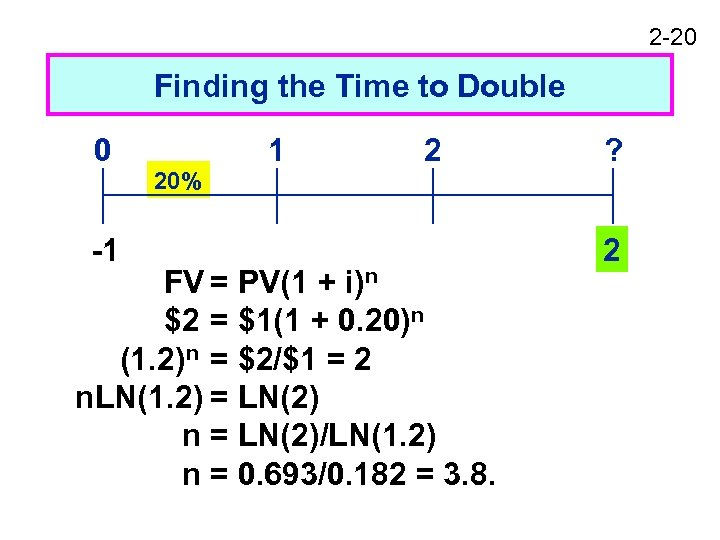2 -20 Finding the Time to Double 0 -1 20% 1 2 FV = PV(1 + i)n \$2 = \$1(1 + 0. 20)n (1. 2)n = \$2/\$1 = 2 n. LN(1. 2) = LN(2) n = LN(2)/LN(1. 2) n = 0. 693/0. 182 = 3. 8. ? 2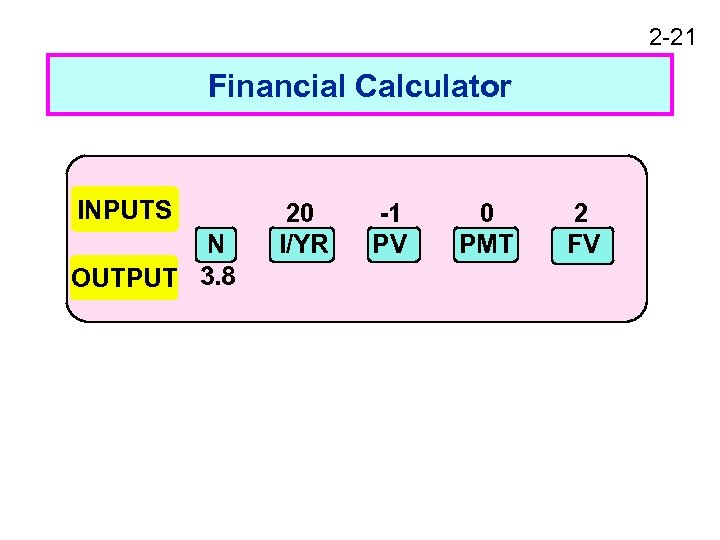2 -21 Financial Calculator INPUTS N OUTPUT 3. 8 20 I/YR -1 PV 0 PMT 2 FV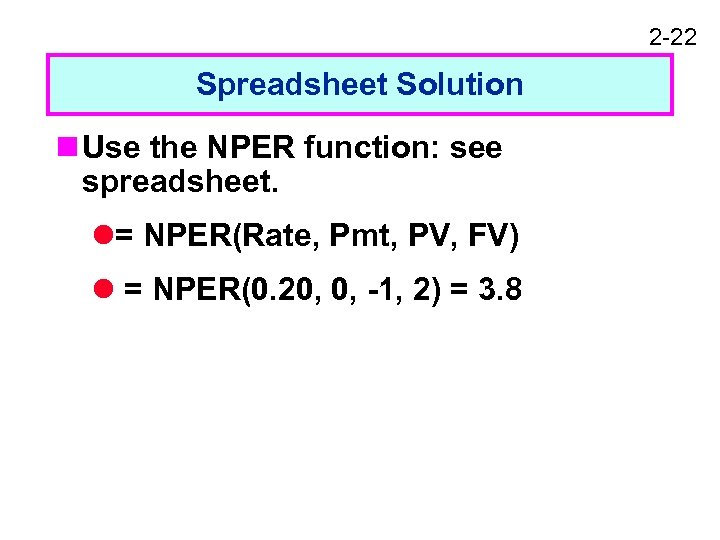2 -22 Spreadsheet Solution n Use the NPER function: see spreadsheet. l= NPER(Rate, Pmt, PV, FV) l = NPER(0. 20, 0, -1, 2) = 3. 8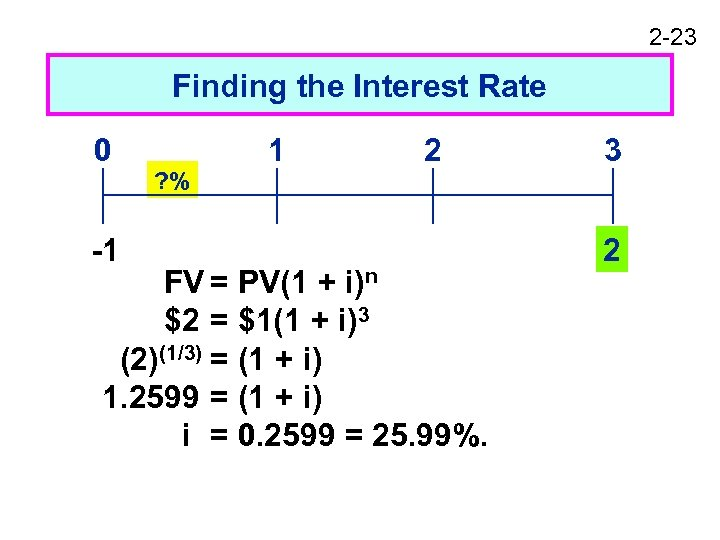2 -23 Finding the Interest Rate 0 -1 ? % 1 2 FV = PV(1 + i)n \$2 = \$1(1 + i)3 (2)(1/3) = (1 + i) 1. 2599 = (1 + i) i = 0. 2599 = 25. 99%. 3 2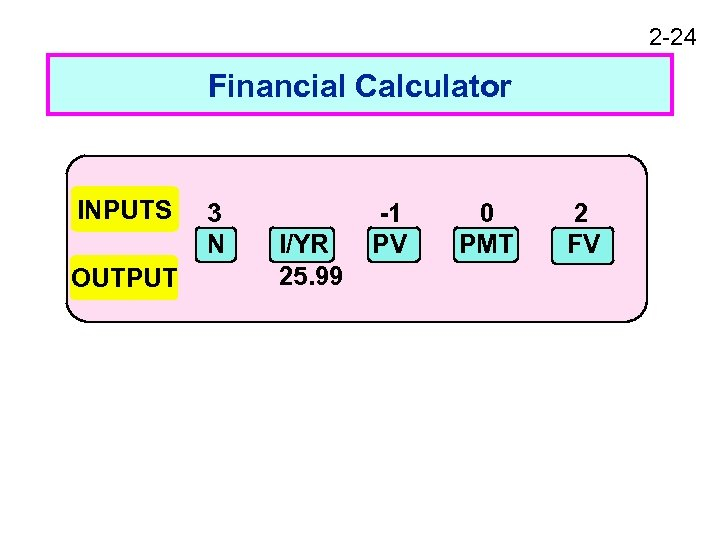2 -24 Financial Calculator INPUTS OUTPUT 3 N I/YR 25. 99 -1 PV 0 PMT 2 FV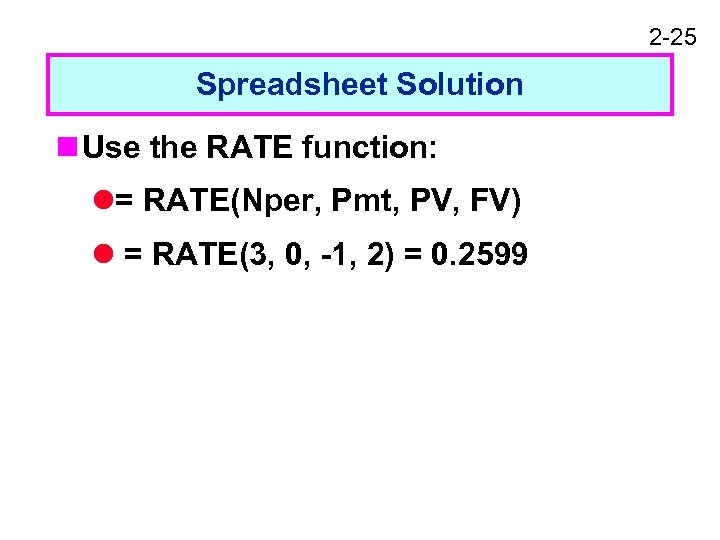2 -25 Spreadsheet Solution n Use the RATE function: l= RATE(Nper, Pmt, PV, FV) l = RATE(3, 0, -1, 2) = 0. 2599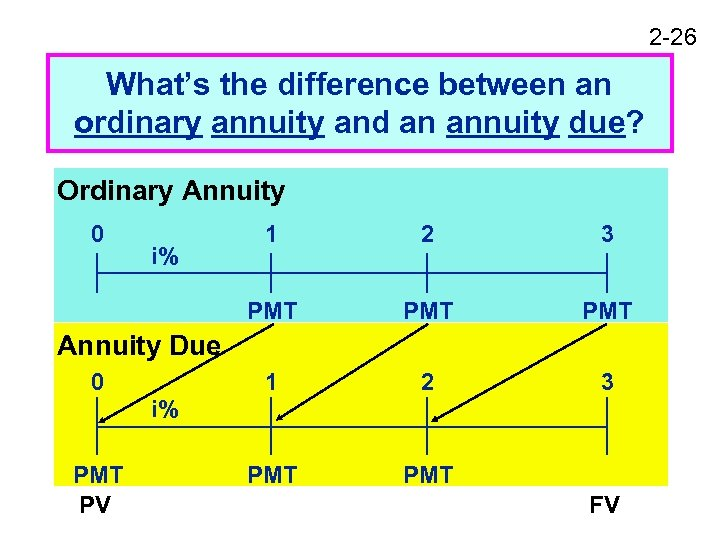2 -26 What’s the difference between an ordinary annuity and an annuity due? Ordinary Annuity 0 2 3 PMT PMT 1 i% 1 2 3 PMT Annuity Due 0 i% PMT PV FV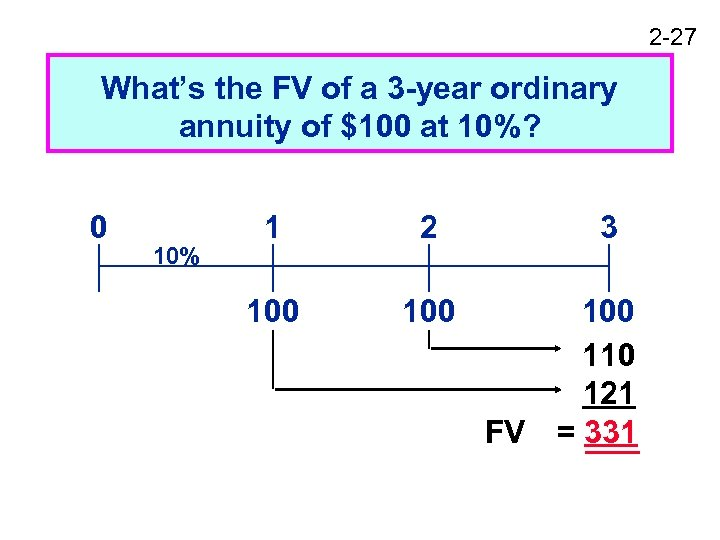2 -27 What’s the FV of a 3 -year ordinary annuity of \$100 at 10%? 0 10% 1 2 100 3 100 110 121 FV = 331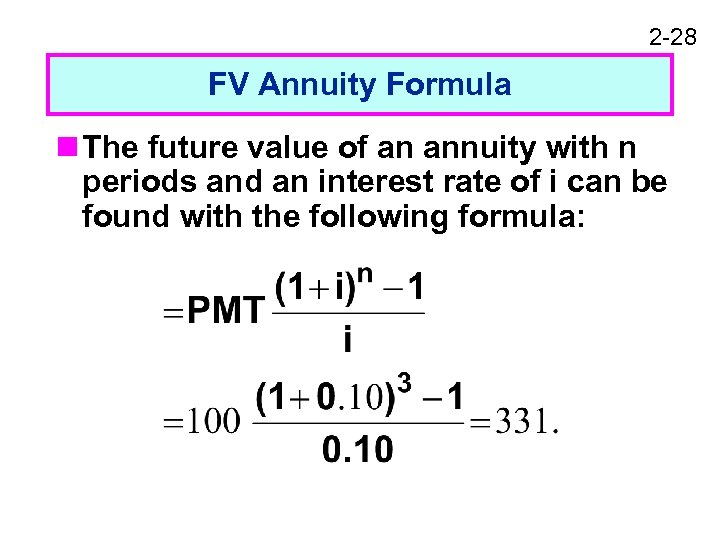2 -28 FV Annuity Formula n The future value of an annuity with n periods and an interest rate of i can be found with the following formula: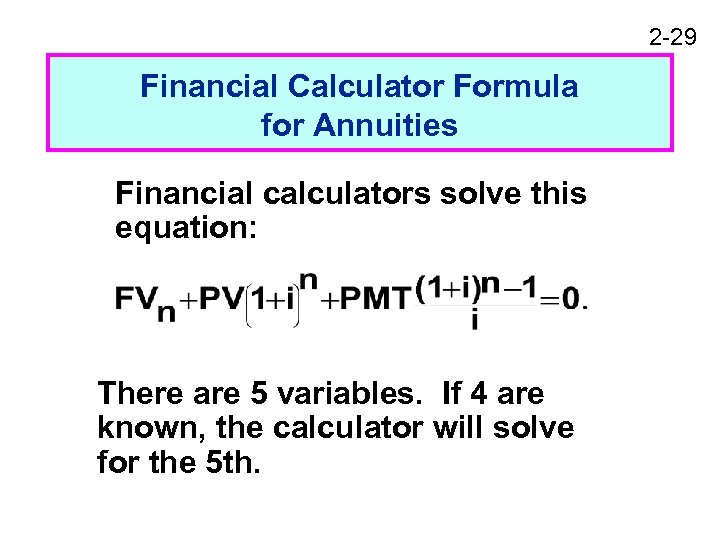2 -29 Financial Calculator Formula for Annuities Financial calculators solve this equation: There are 5 variables. If 4 are known, the calculator will solve for the 5 th.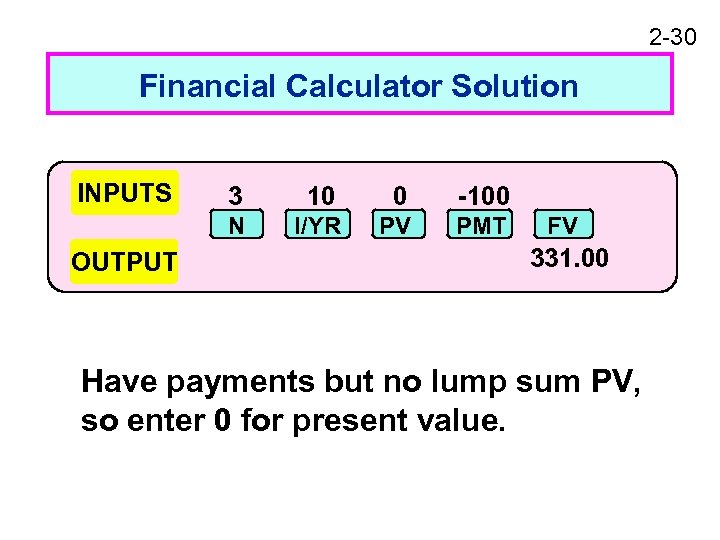2 -30 Financial Calculator Solution INPUTS 10 0 -100 N OUTPUT 3 I/YR PV PMT FV 331. 00 Have payments but no lump sum PV, so enter 0 for present value.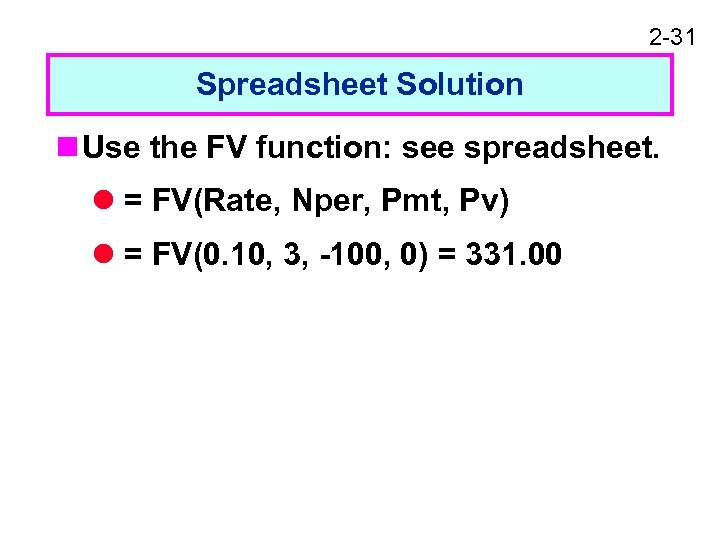2 -31 Spreadsheet Solution n Use the FV function: see spreadsheet. l = FV(Rate, Nper, Pmt, Pv) l = FV(0. 10, 3, -100, 0) = 331. 00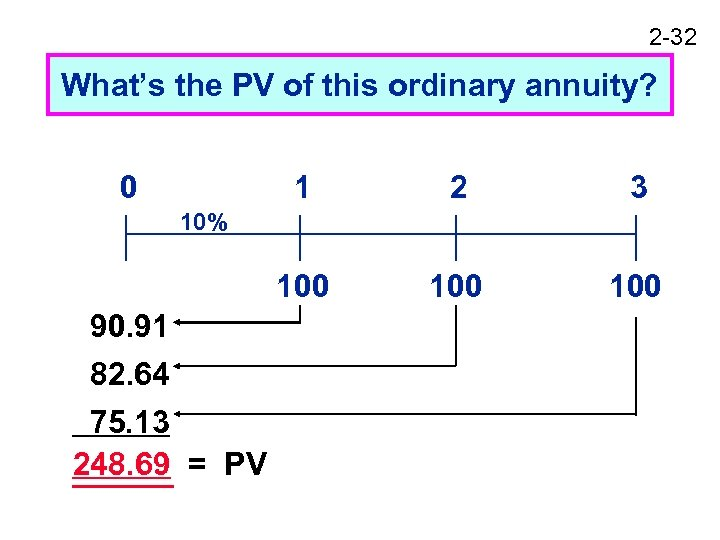2 -32 What’s the PV of this ordinary annuity? 0 1 2 3 100 100 10% 90. 91 82. 64 75. 13 248. 69 = PV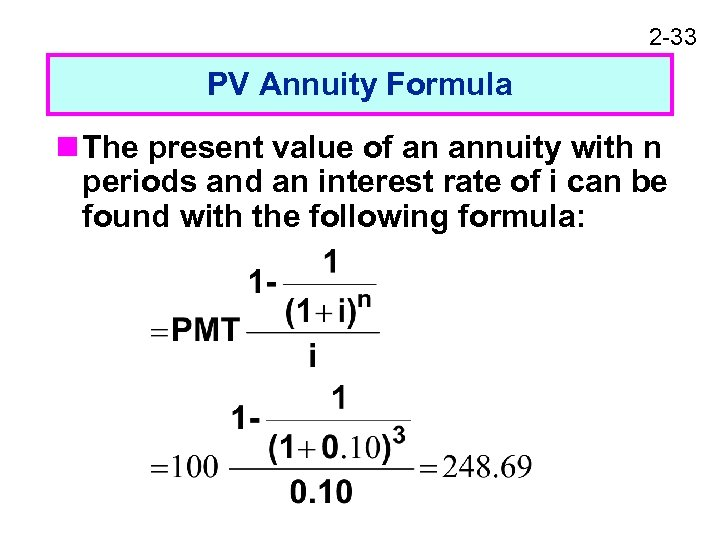2 -33 PV Annuity Formula n The present value of an annuity with n periods and an interest rate of i can be found with the following formula: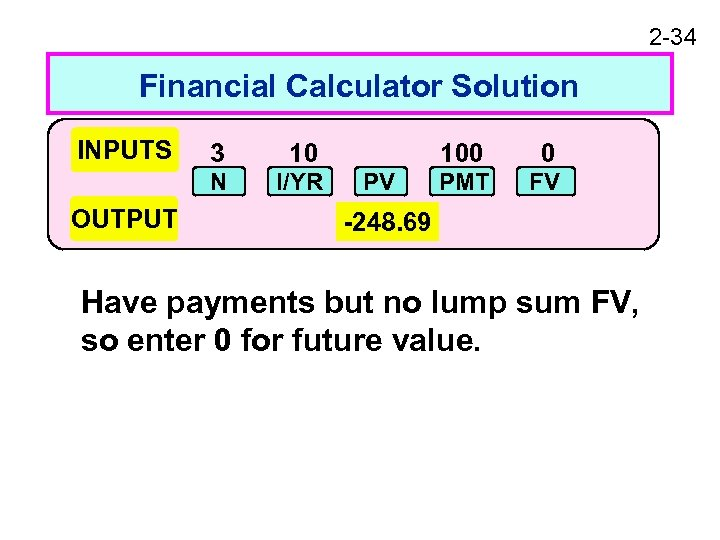2 -34 Financial Calculator Solution INPUTS 10 N OUTPUT 3 I/YR 100 PV 0 PMT FV -248. 69 Have payments but no lump sum FV, so enter 0 for future value.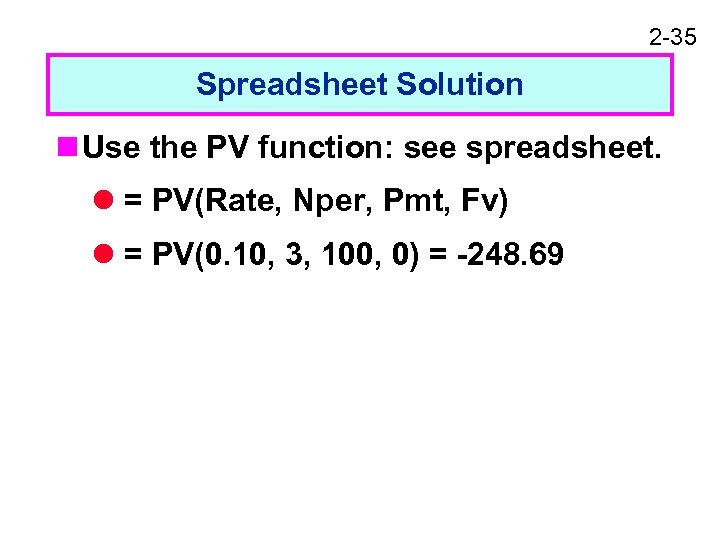2 -35 Spreadsheet Solution n Use the PV function: see spreadsheet. l = PV(Rate, Nper, Pmt, Fv) l = PV(0. 10, 3, 100, 0) = -248. 69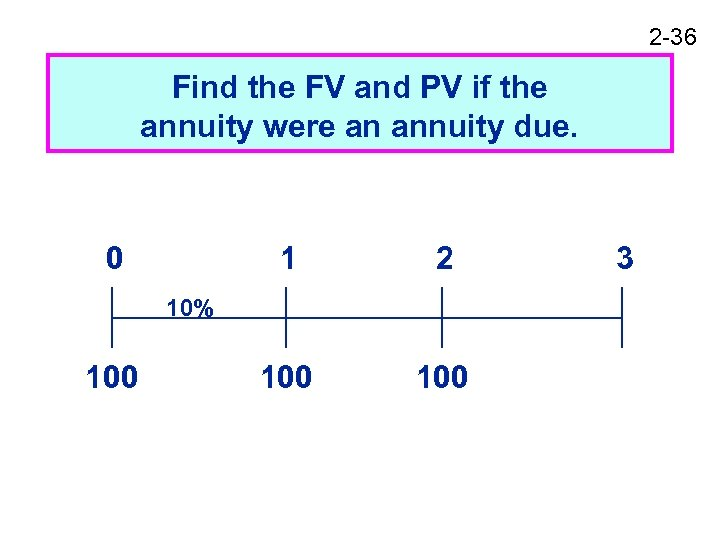2 -36 Find the FV and PV if the annuity were an annuity due. 0 1 2 100 10% 100 3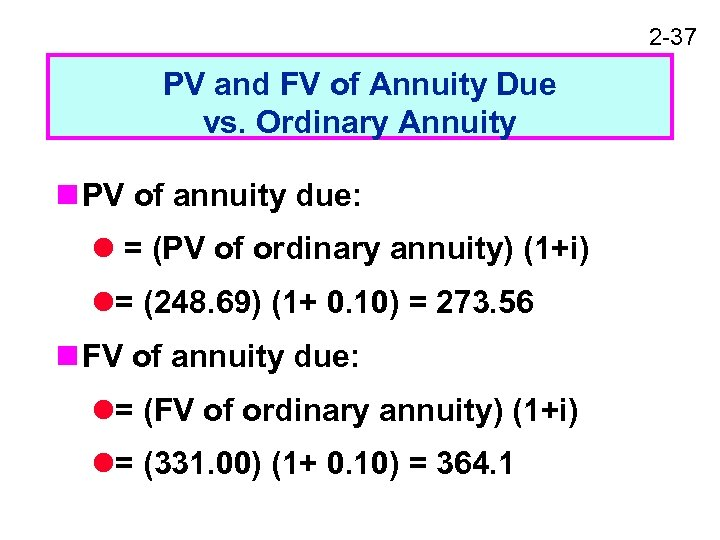2 -37 PV and FV of Annuity Due vs. Ordinary Annuity n PV of annuity due: l = (PV of ordinary annuity) (1+i) l= (248. 69) (1+ 0. 10) = 273. 56 n FV of annuity due: l= (FV of ordinary annuity) (1+i) l= (331. 00) (1+ 0. 10) = 364. 1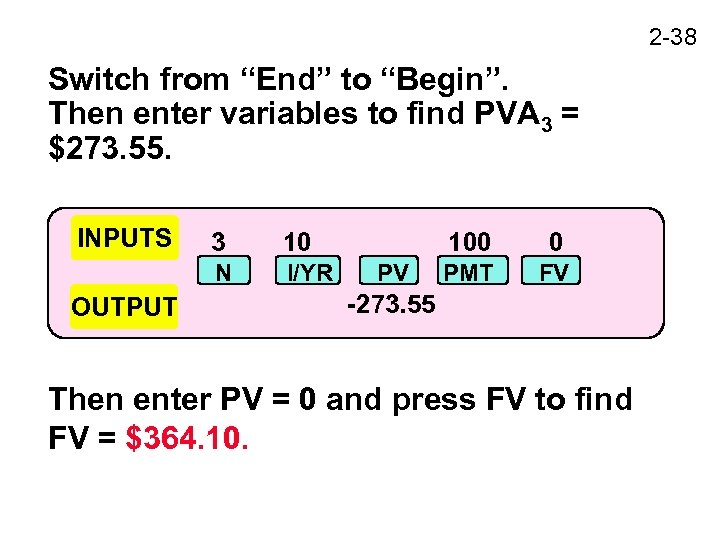2 -38 Switch from “End” to “Begin”. Then enter variables to find PVA 3 = \$273. 55. INPUTS 10 N OUTPUT 3 I/YR 100 PV 0 PMT FV -273. 55 Then enter PV = 0 and press FV to find FV = \$364. 10.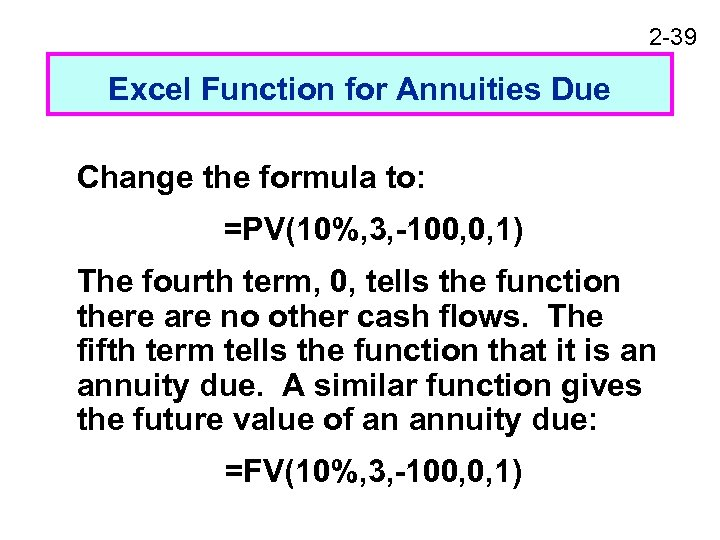2 -39 Excel Function for Annuities Due Change the formula to: =PV(10%, 3, -100, 0, 1) The fourth term, 0, tells the function there are no other cash flows. The fifth term tells the function that it is an annuity due. A similar function gives the future value of an annuity due: =FV(10%, 3, -100, 0, 1)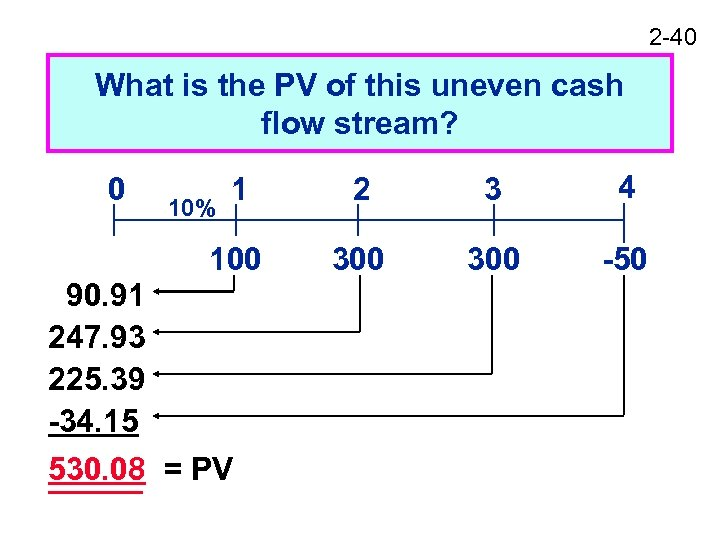2 -40 What is the PV of this uneven cash flow stream? 1 2 3 4 100 0 300 -50 10% 90. 91 247. 93 225. 39 -34. 15 530. 08 = PV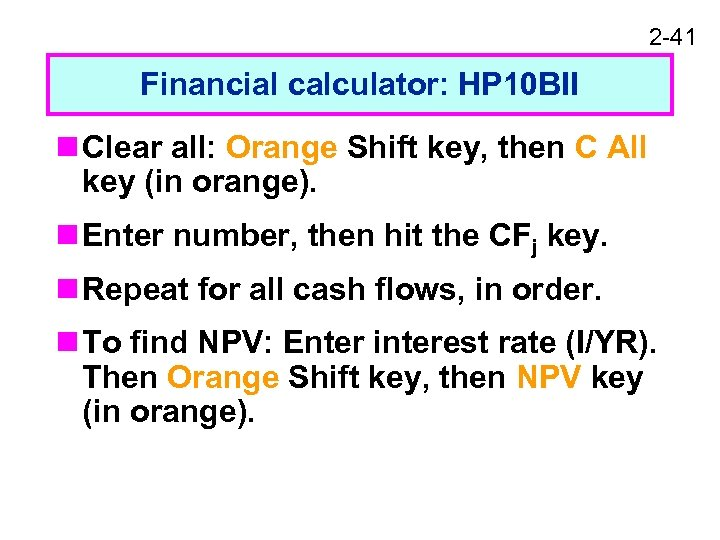2 -41 Financial calculator: HP 10 BII n Clear all: Orange Shift key, then C All key (in orange). n Enter number, then hit the CFj key. n Repeat for all cash flows, in order. n To find NPV: Enter interest rate (I/YR). Then Orange Shift key, then NPV key (in orange).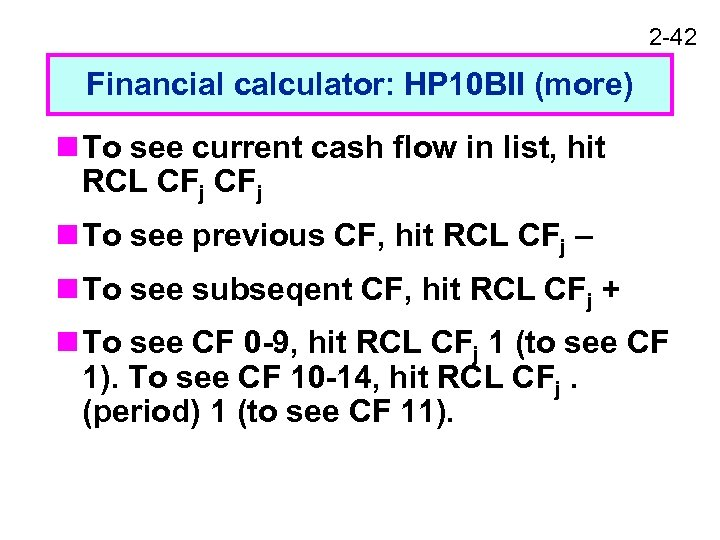2 -42 Financial calculator: HP 10 BII (more) n To see current cash flow in list, hit RCL CFj n To see previous CF, hit RCL CFj – n To see subseqent CF, hit RCL CFj + n To see CF 0 -9, hit RCL CFj 1 (to see CF 1). To see CF 10 -14, hit RCL CFj. (period) 1 (to see CF 11).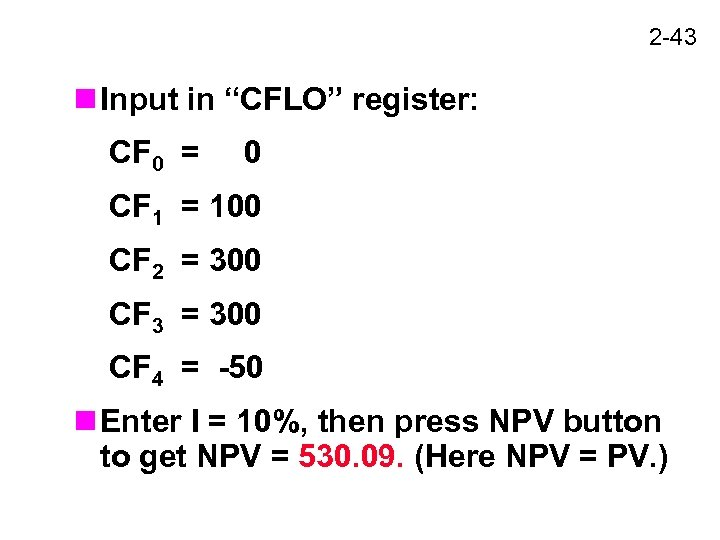2 -43 n Input in “CFLO” register: CF 0 = 0 CF 1 = 100 CF 2 = 300 CF 3 = 300 CF 4 = -50 n Enter I = 10%, then press NPV button to get NPV = 530. 09. (Here NPV = PV. )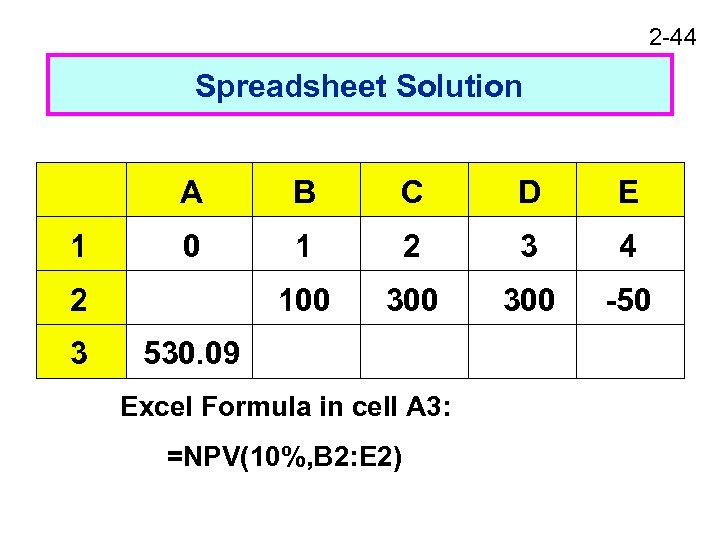2 -44 Spreadsheet Solution A 1 B C D E 0 1 2 3 4 100 300 -50 2 3 530. 09 Excel Formula in cell A 3: =NPV(10%, B 2: E 2)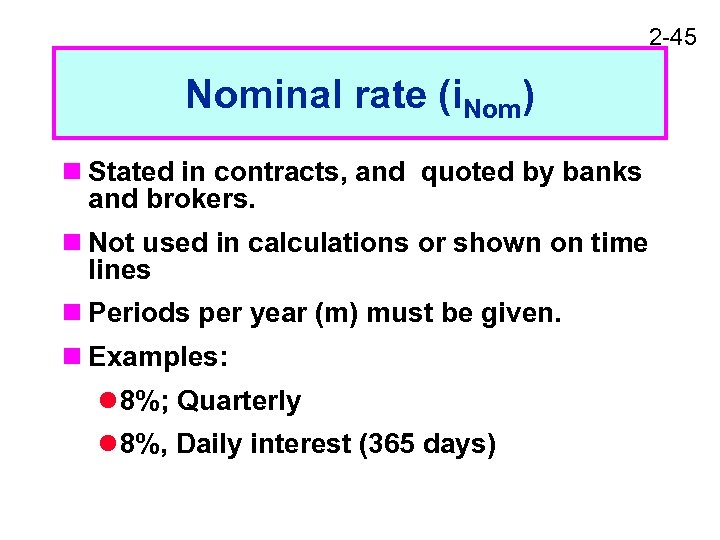2 -45 Nominal rate (i. Nom) n Stated in contracts, and quoted by banks and brokers. n Not used in calculations or shown on time lines n Periods per year (m) must be given. n Examples: l 8%; Quarterly l 8%, Daily interest (365 days)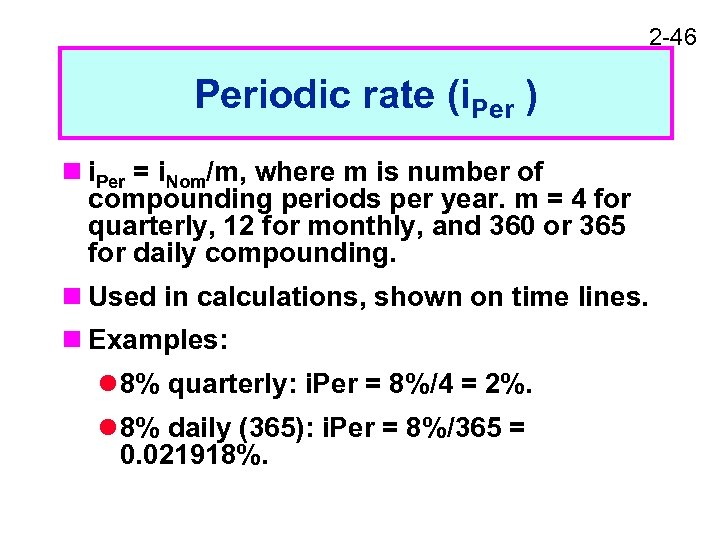2 -46 Periodic rate (i. Per ) n i. Per = i. Nom/m, where m is number of compounding periods per year. m = 4 for quarterly, 12 for monthly, and 360 or 365 for daily compounding. n Used in calculations, shown on time lines. n Examples: l 8% quarterly: i. Per = 8%/4 = 2%. l 8% daily (365): i. Per = 8%/365 = 0. 021918%.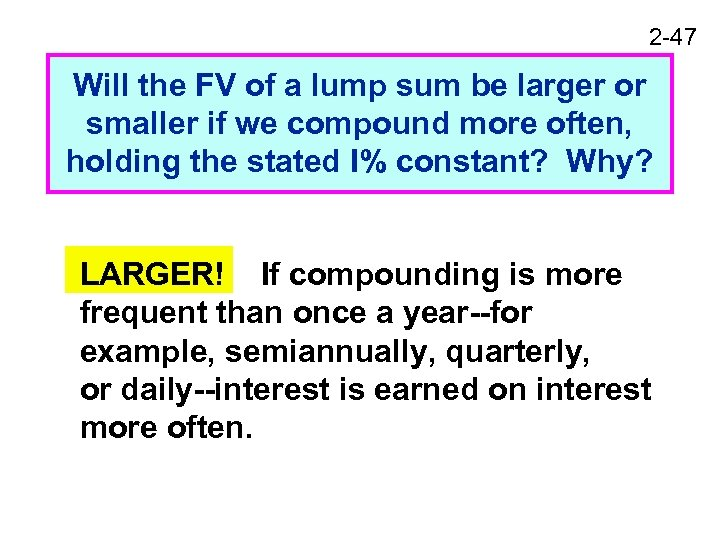2 -47 Will the FV of a lump sum be larger or smaller if we compound more often, holding the stated I% constant? Why? LARGER! If compounding is more frequent than once a year--for example, semiannually, quarterly, or daily--interest is earned on interest more often.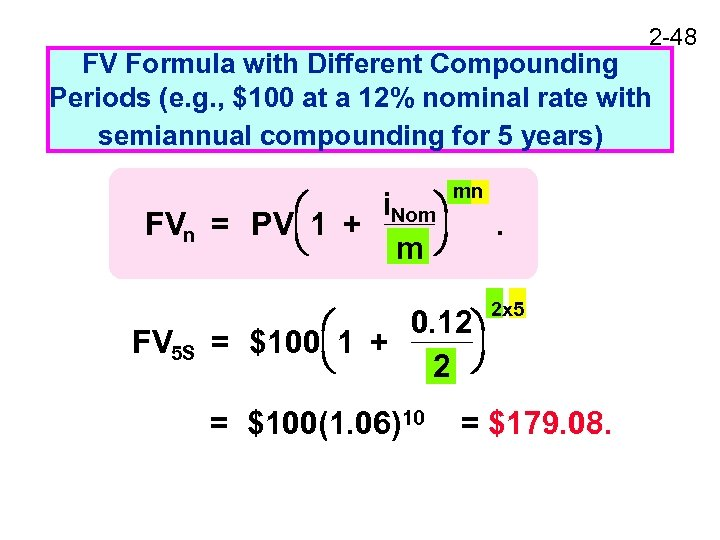2 -48 FV Formula with Different Compounding Periods (e. g. , \$100 at a 12% nominal rate with semiannual compounding for 5 years) 1 + i. Nom FVn = PV m FV 5 S mn . 1 + 0. 12 = \$100 2 = \$100(1. 06)10 2 x 5 = \$179. 08.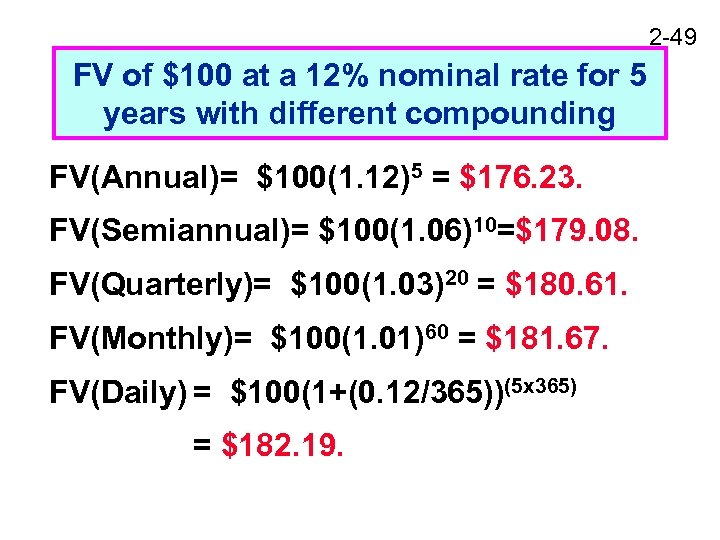2 -49 FV of \$100 at a 12% nominal rate for 5 years with different compounding FV(Annual)= \$100(1. 12)5 = \$176. 23. FV(Semiannual)= \$100(1. 06)10=\$179. 08. FV(Quarterly)= \$100(1. 03)20 = \$180. 61. FV(Monthly)= \$100(1. 01)60 = \$181. 67. FV(Daily) = \$100(1+(0. 12/365))(5 x 365) = \$182. 19.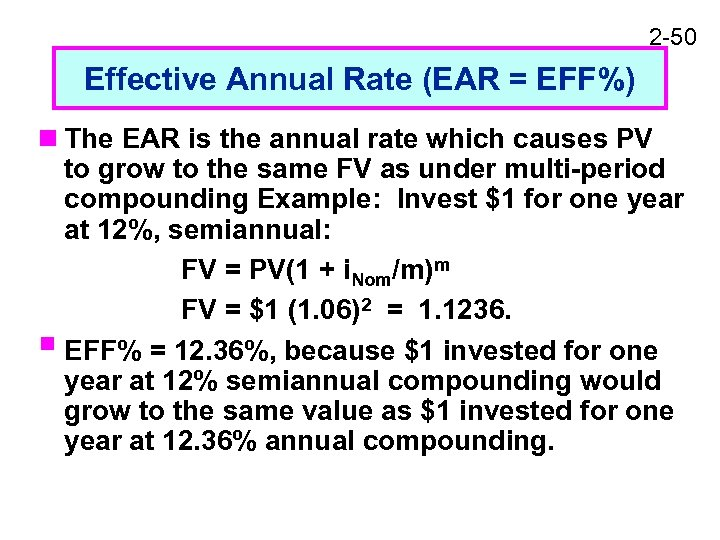2 -50 Effective Annual Rate (EAR = EFF%) n The EAR is the annual rate which causes PV to grow to the same FV as under multi-period compounding Example: Invest \$1 for one year at 12%, semiannual: FV = PV(1 + i. Nom/m)m FV = \$1 (1. 06)2 = 1. 1236. § EFF% = 12. 36%, because \$1 invested for one year at 12% semiannual compounding would grow to the same value as \$1 invested for one year at 12. 36% annual compounding.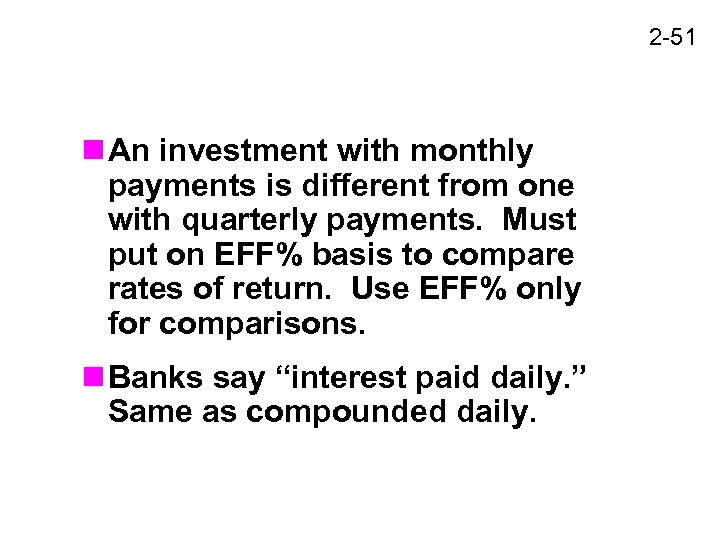2 -51 n An investment with monthly payments is different from one with quarterly payments. Must put on EFF% basis to compare rates of return. Use EFF% only for comparisons. n Banks say “interest paid daily. ” Same as compounded daily.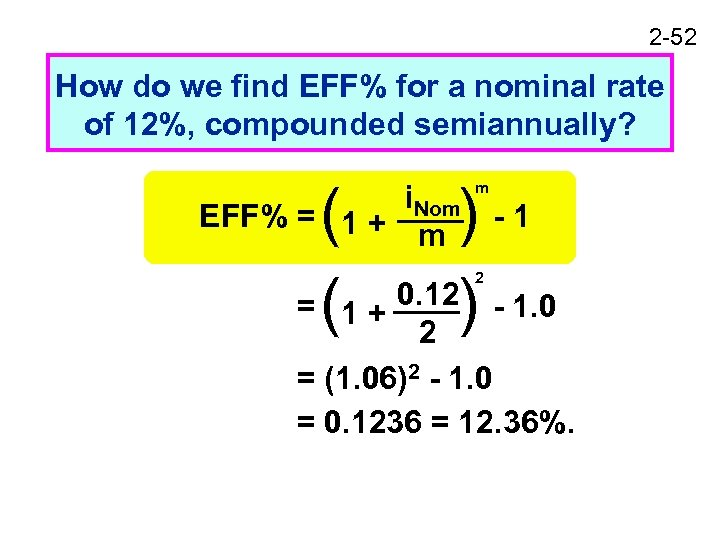2 -52 How do we find EFF% for a nominal rate of 12%, compounded semiannually? ( ) -1 = (1 + 0. 12) - 1. 0 2 i. Nom EFF% = 1 + m m 2 = (1. 06)2 - 1. 0 = 0. 1236 = 12. 36%.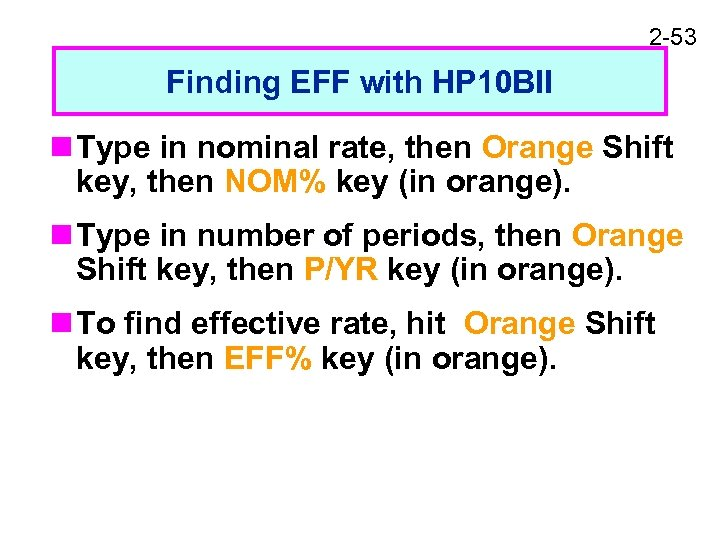2 -53 Finding EFF with HP 10 BII n Type in nominal rate, then Orange Shift key, then NOM% key (in orange). n Type in number of periods, then Orange Shift key, then P/YR key (in orange). n To find effective rate, hit Orange Shift key, then EFF% key (in orange).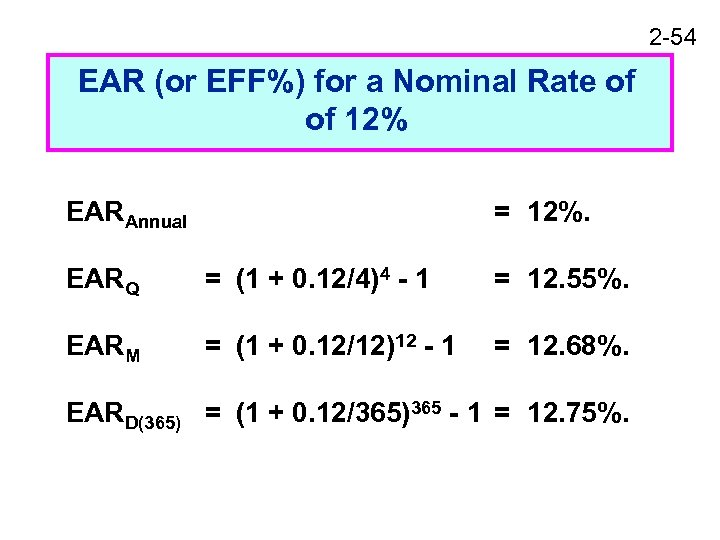2 -54 EAR (or EFF%) for a Nominal Rate of of 12% EARAnnual = 12%. EARQ = (1 + 0. 12/4)4 - 1 = 12. 55%. EARM = (1 + 0. 12/12)12 - 1 = 12. 68%. EARD(365) = (1 + 0. 12/365)365 - 1 = 12. 75%.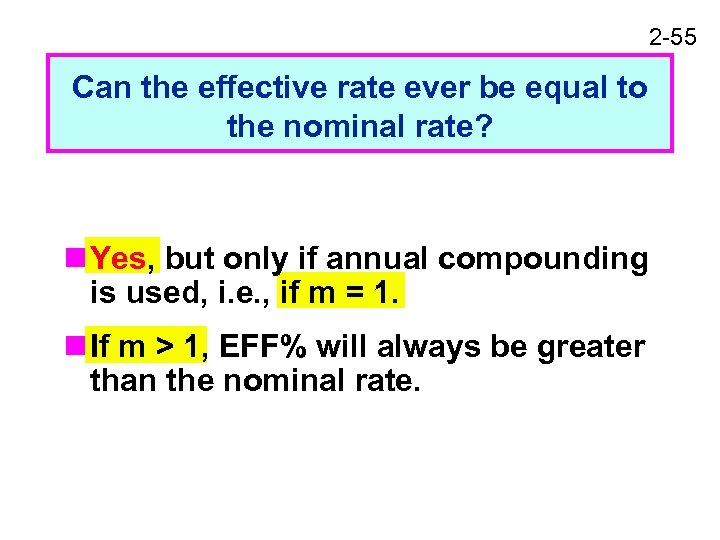2 -55 Can the effective rate ever be equal to the nominal rate? n Yes, but only if annual compounding is used, i. e. , if m = 1. n If m > 1, EFF% will always be greater than the nominal rate.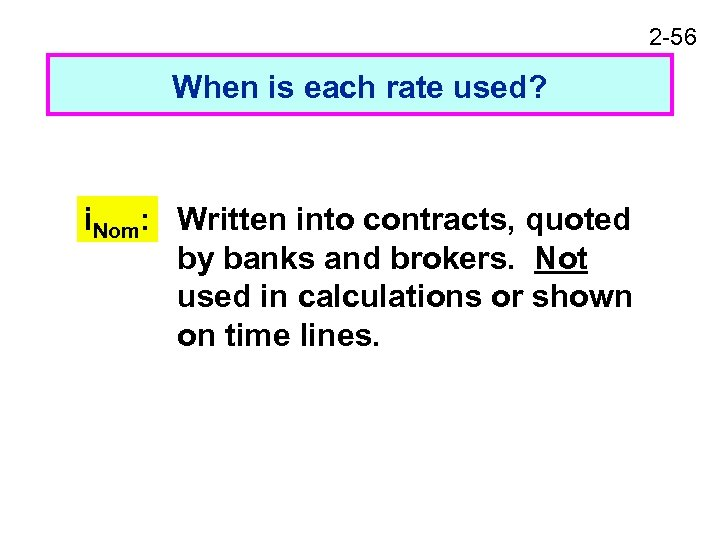2 -56 When is each rate used? i. Nom: Written into contracts, quoted by banks and brokers. Not used in calculations or shown on time lines.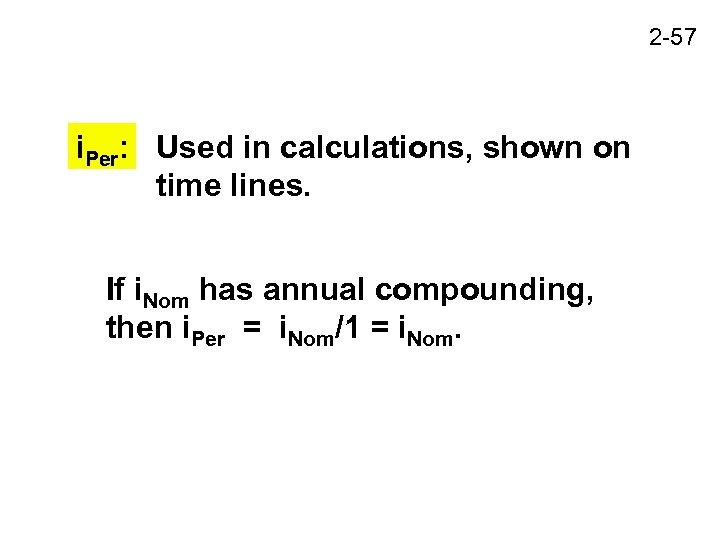2 -57 i. Per: Used in calculations, shown on time lines. If i. Nom has annual compounding, then i. Per = i. Nom/1 = i. Nom.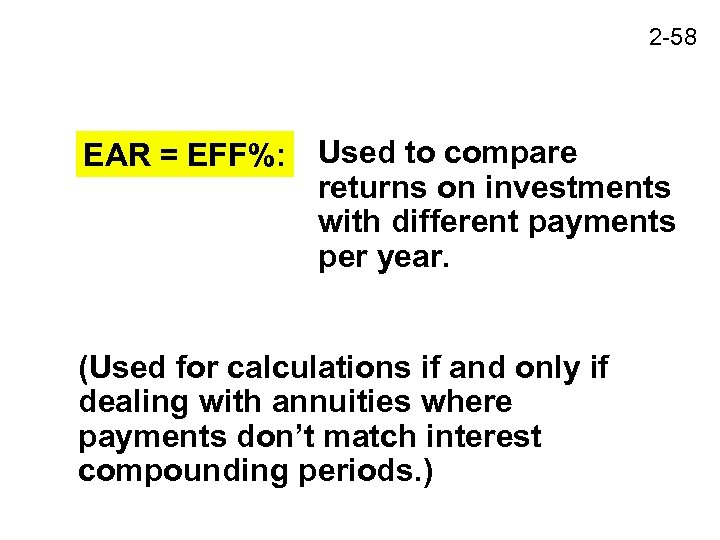2 -58 EAR = EFF%: Used to compare returns on investments with different payments per year. (Used for calculations if and only if dealing with annuities where payments don’t match interest compounding periods. )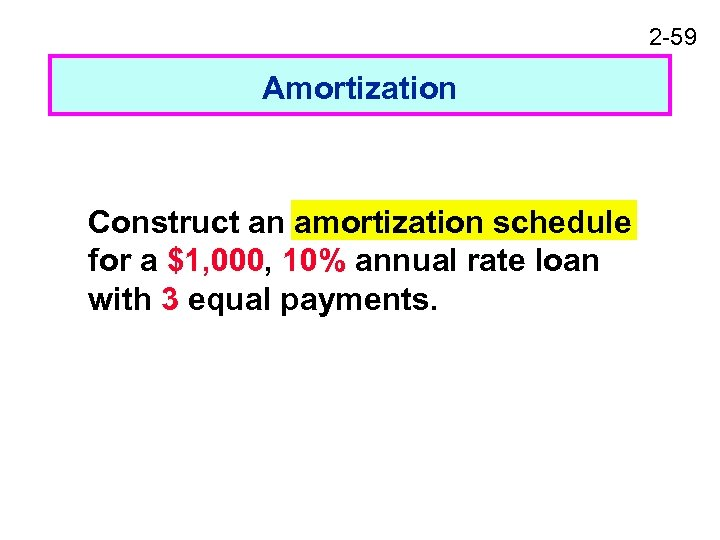2 -59 Amortization Construct an amortization schedule for a \$1, 000, 10% annual rate loan with 3 equal payments.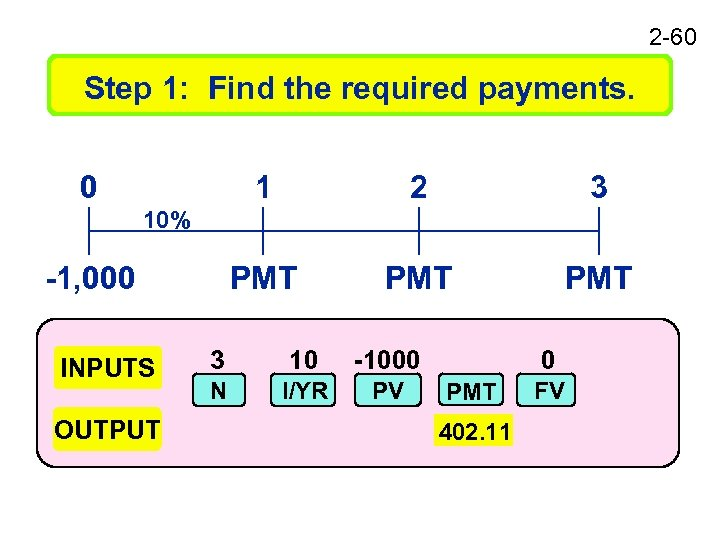2 -60 Step 1: Find the required payments. 0 1 2 3 PMT PMT 10% -1, 000 INPUTS OUTPUT 3 10 -1000 N I/YR PV 0 PMT 402. 11 FV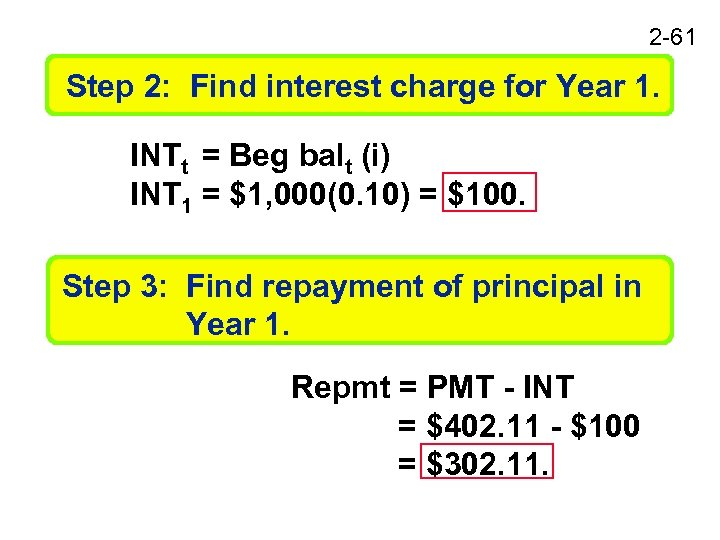2 -61 Step 2: Find interest charge for Year 1. INTt = Beg balt (i) INT 1 = \$1, 000(0. 10) = \$100. Step 3: Find repayment of principal in Year 1. Repmt = PMT - INT = \$402. 11 - \$100 = \$302. 11.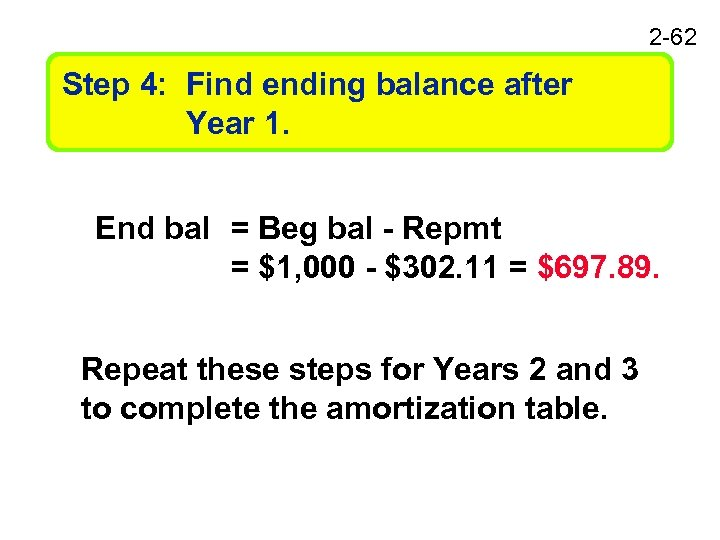2 -62 Step 4: Find ending balance after Year 1. End bal = Beg bal - Repmt = \$1, 000 - \$302. 11 = \$697. 89. Repeat these steps for Years 2 and 3 to complete the amortization table.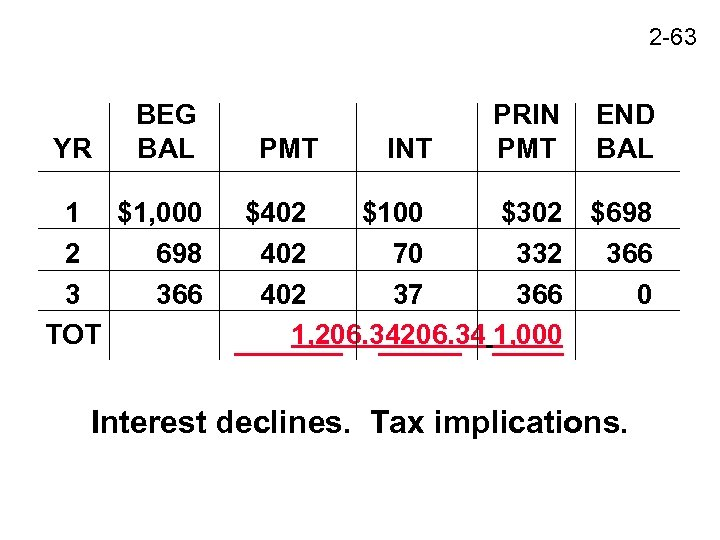2 -63 YR BEG BAL 1 \$1, 000 2 698 3 366 TOT PMT INT PRIN PMT END BAL \$402 \$100 \$302 \$698 402 70 332 366 402 37 366 0 1, 206. 34 1, 000 Interest declines. Tax implications.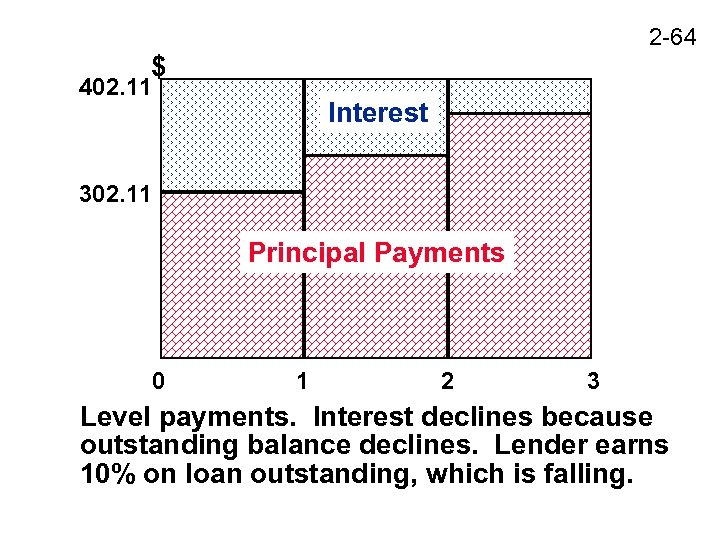2 -64 \$ 402. 11 Interest 302. 11 Principal Payments 0 1 2 3 Level payments. Interest declines because outstanding balance declines. Lender earns 10% on loan outstanding, which is falling.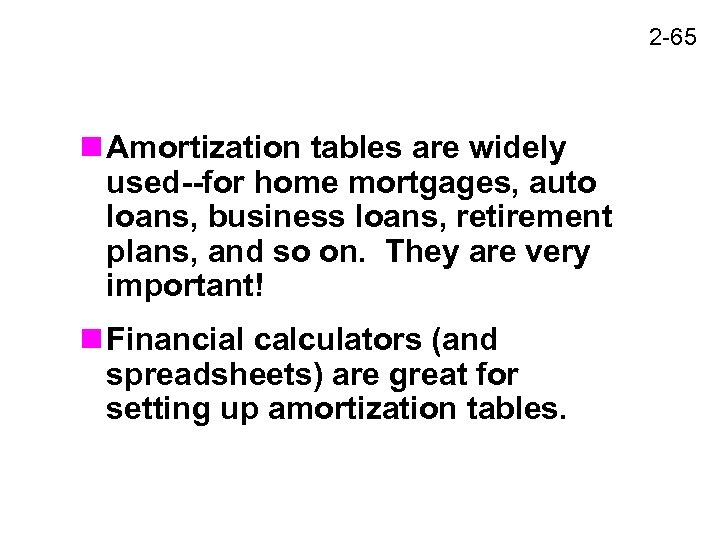2 -65 n Amortization tables are widely used--for home mortgages, auto loans, business loans, retirement plans, and so on. They are very important! n Financial calculators (and spreadsheets) are great for setting up amortization tables.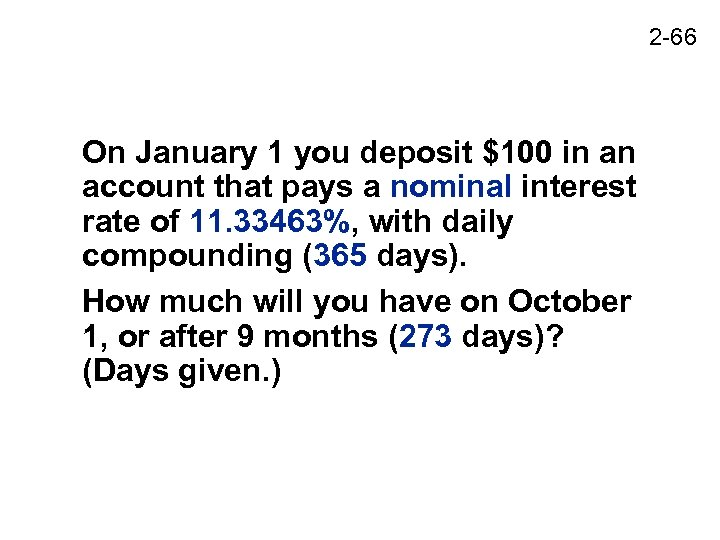2 -66 On January 1 you deposit \$100 in an account that pays a nominal interest rate of 11. 33463%, with daily compounding (365 days). How much will you have on October 1, or after 9 months (273 days)? (Days given. )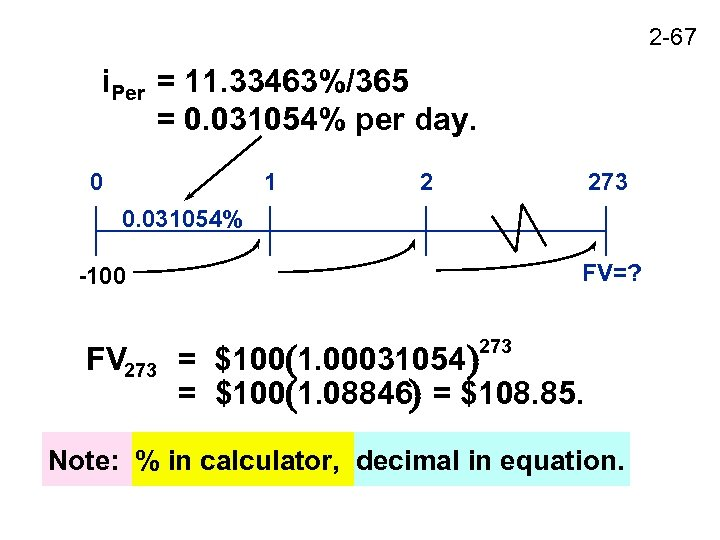2 -67 i. Per = 11. 33463%/365 = 0. 031054% per day. 0 1 2 273 0. 031054% FV=? -100 FV 273 = \$100 1. 00031054 = \$100 1. 08846 = \$108. 85. 273 Note: % in calculator, decimal in equation.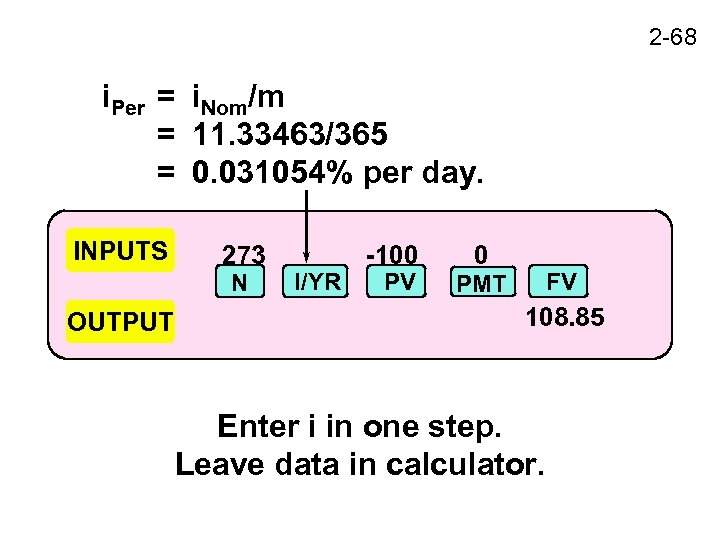2 -68 i. Per = i. Nom/m = 11. 33463/365 = 0. 031054% per day. INPUTS 273 N OUTPUT I/YR -100 PV 0 FV PMT 108. 85 Enter i in one step. Leave data in calculator.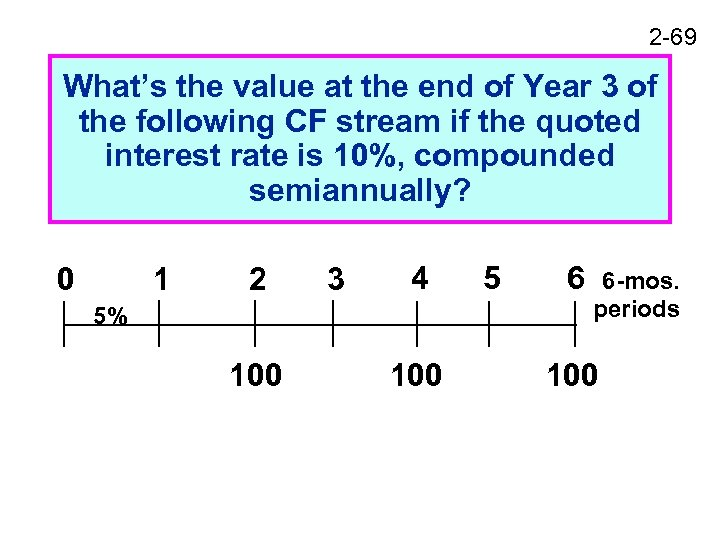2 -69 What’s the value at the end of Year 3 of the following CF stream if the quoted interest rate is 10%, compounded semiannually? 0 1 2 3 4 5% 100 5 6 6 -mos. periods 100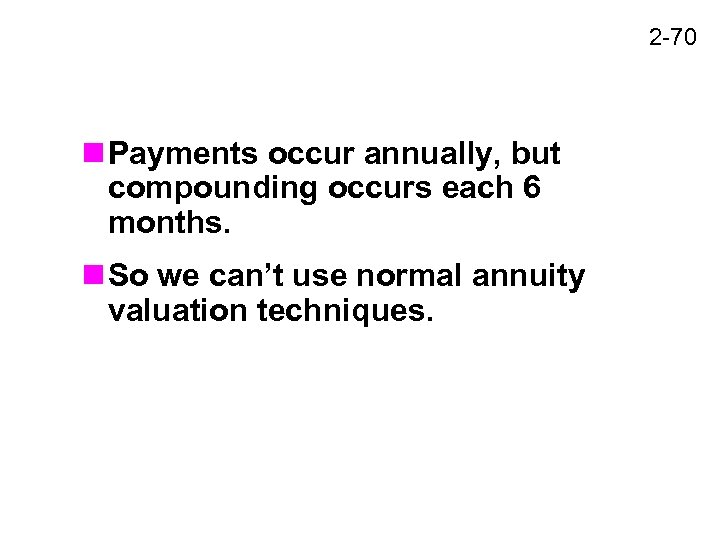2 -70 n Payments occur annually, but compounding occurs each 6 months. n So we can’t use normal annuity valuation techniques.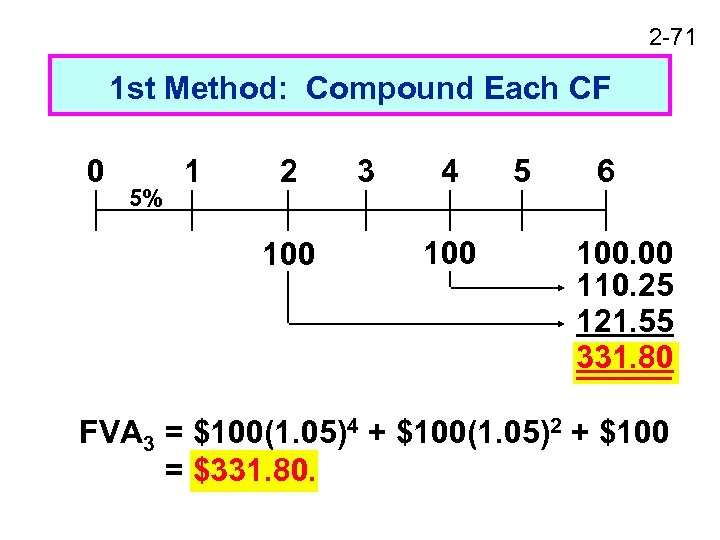2 -71 1 st Method: Compound Each CF 0 5% 1 2 100 3 4 100 5 6 100. 00 110. 25 121. 55 331. 80 FVA 3 = \$100(1. 05)4 + \$100(1. 05)2 + \$100 = \$331. 80.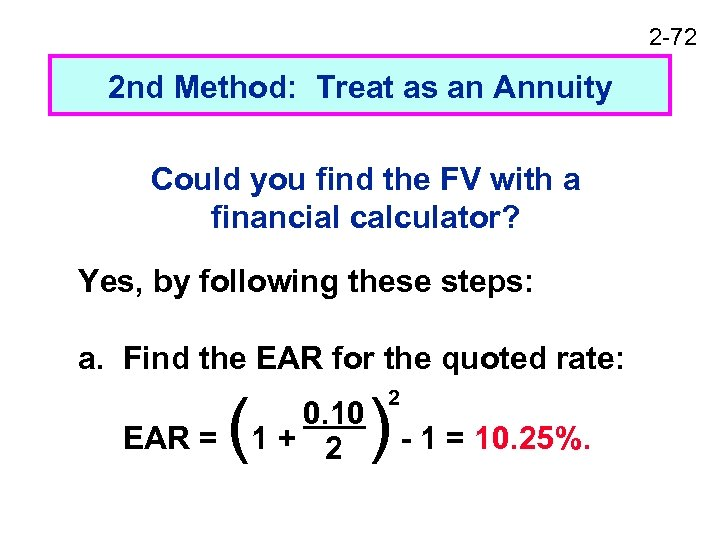2 -72 2 nd Method: Treat as an Annuity Could you find the FV with a financial calculator? Yes, by following these steps: a. Find the EAR for the quoted rate: EAR = ( 0. 10 1+ 2 ) - 1 = 10. 25%. 2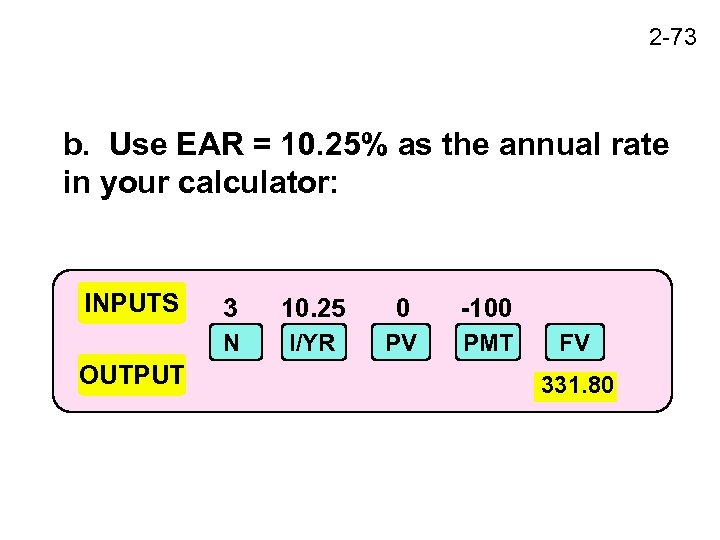2 -73 b. Use EAR = 10. 25% as the annual rate in your calculator: INPUTS 10. 25 0 -100 N OUTPUT 3 I/YR PV PMT FV 331. 80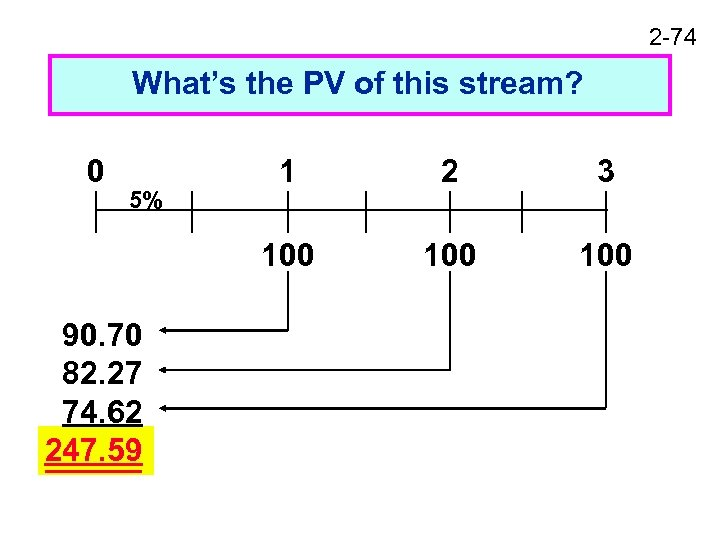2 -74 What’s the PV of this stream? 0 90. 70 82. 27 74. 62 247. 59 2 3 100 5% 1 100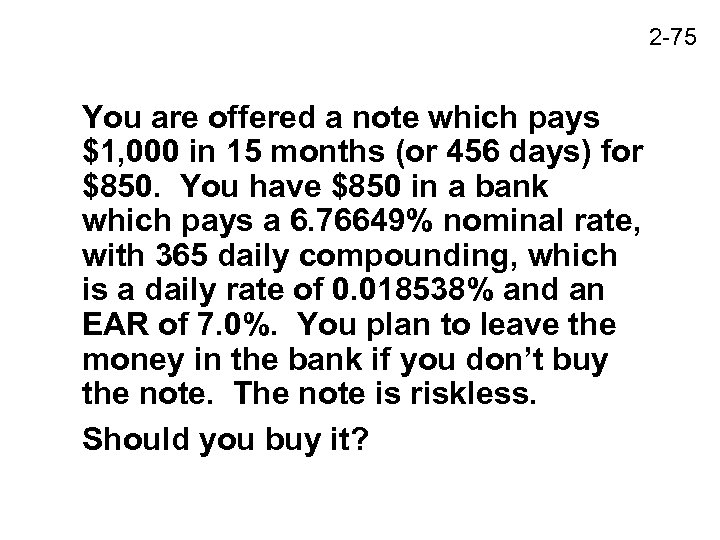2 -75 You are offered a note which pays \$1, 000 in 15 months (or 456 days) for \$850. You have \$850 in a bank which pays a 6. 76649% nominal rate, with 365 daily compounding, which is a daily rate of 0. 018538% and an EAR of 7. 0%. You plan to leave the money in the bank if you don’t buy the note. The note is riskless. Should you buy it?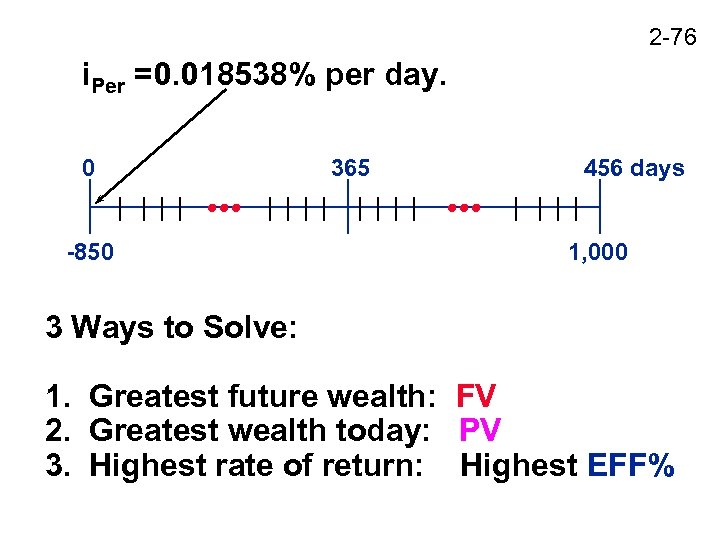2 -76 i. Per =0. 018538% per day. 0 -850 365 456 days 1, 000 3 Ways to Solve: 1. Greatest future wealth: FV 2. Greatest wealth today: PV 3. Highest rate of return: Highest EFF%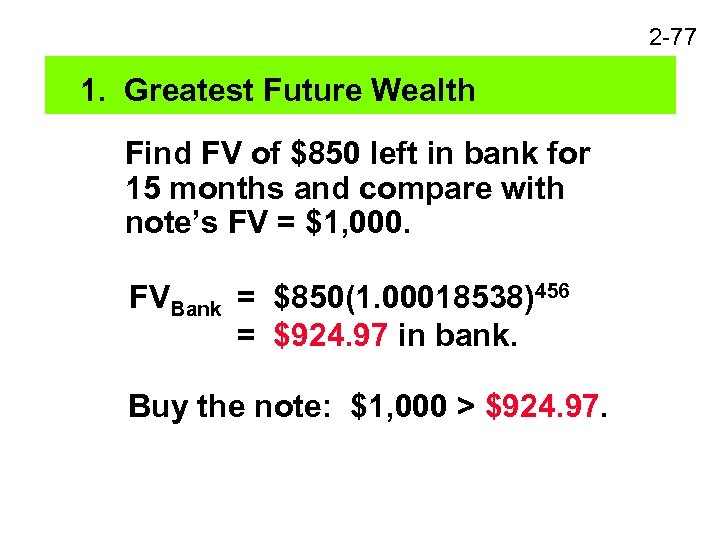2 -77 1. Greatest Future Wealth Find FV of \$850 left in bank for 15 months and compare with note’s FV = \$1, 000. FVBank = \$850(1. 00018538)456 = \$924. 97 in bank. Buy the note: \$1, 000 > \$924. 97.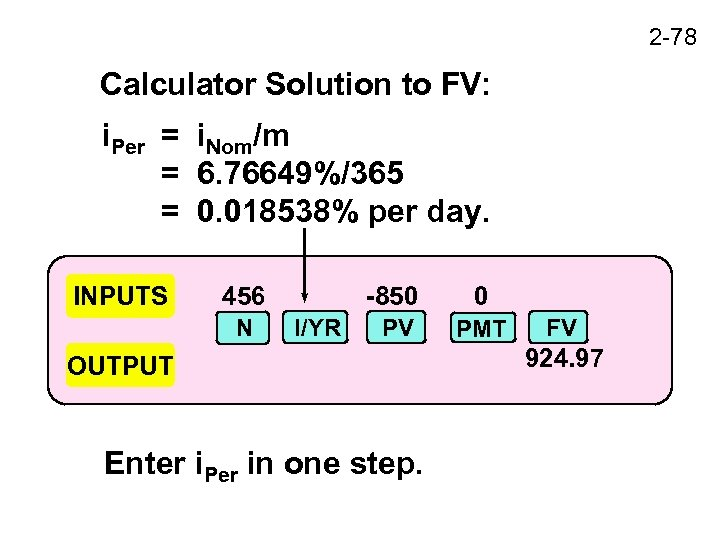2 -78 Calculator Solution to FV: i. Per = i. Nom/m = 6. 76649%/365 = 0. 018538% per day. INPUTS 456 N -850 I/YR 0 PV PMT OUTPUT Enter i. Per in one step. FV 924. 97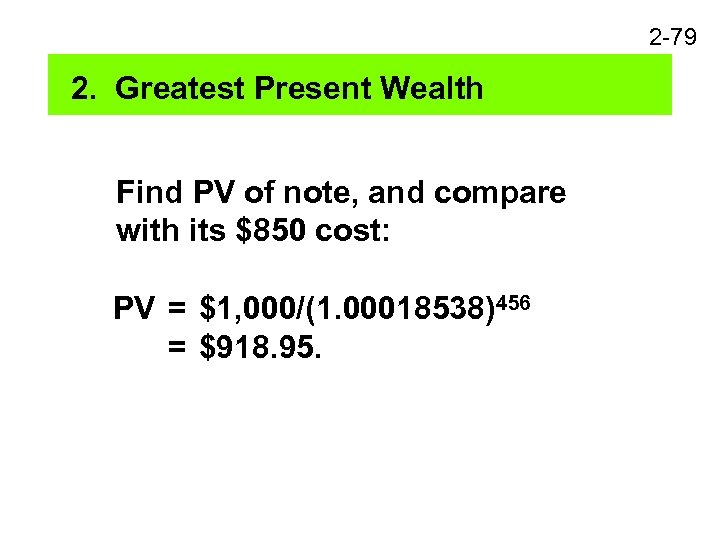2 -79 2. Greatest Present Wealth Find PV of note, and compare with its \$850 cost: PV = \$1, 000/(1. 00018538)456 = \$918. 95.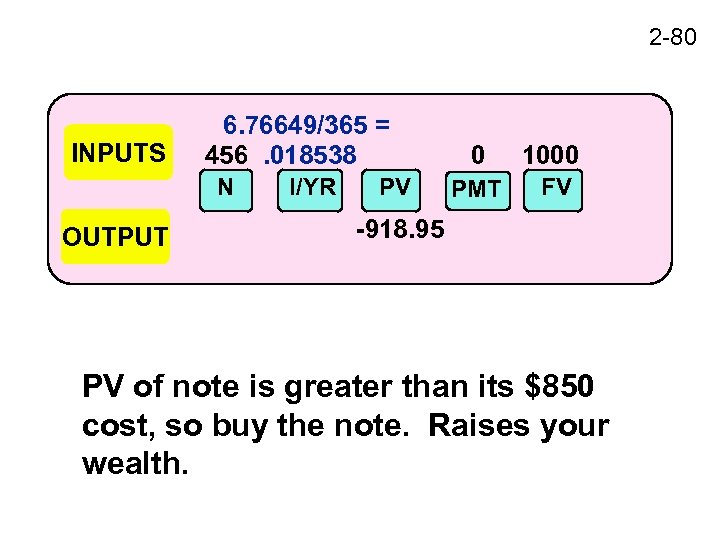2 -80 INPUTS 6. 76649/365 = 456. 018538 N OUTPUT I/YR PV 0 1000 PMT FV -918. 95 PV of note is greater than its \$850 cost, so buy the note. Raises your wealth.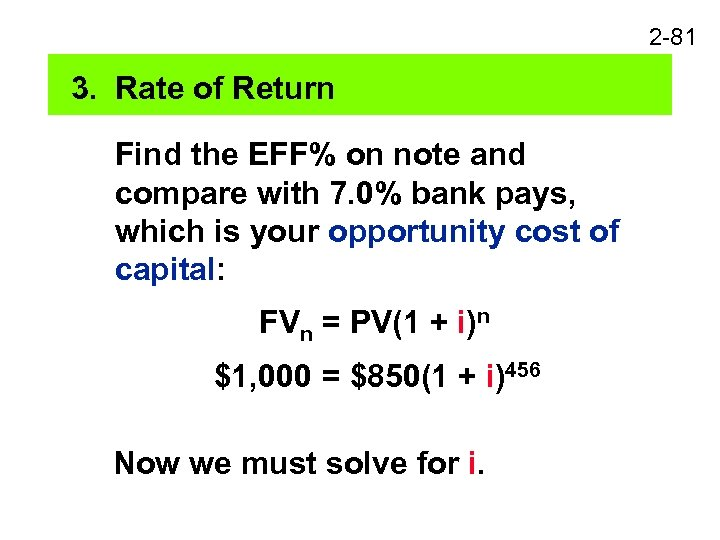2 -81 3. Rate of Return Find the EFF% on note and compare with 7. 0% bank pays, which is your opportunity cost of capital: FVn = PV(1 + i)n \$1, 000 = \$850(1 + i)456 Now we must solve for i.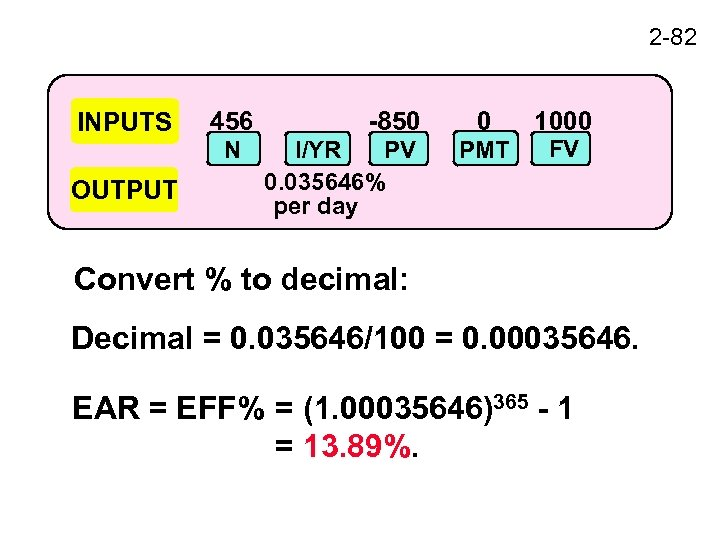2 -82 INPUTS OUTPUT 456 N -850 I/YR PV 0. 035646% per day 0 PMT 1000 FV Convert % to decimal: Decimal = 0. 035646/100 = 0. 00035646. EAR = EFF% = (1. 00035646)365 - 1 = 13. 89%.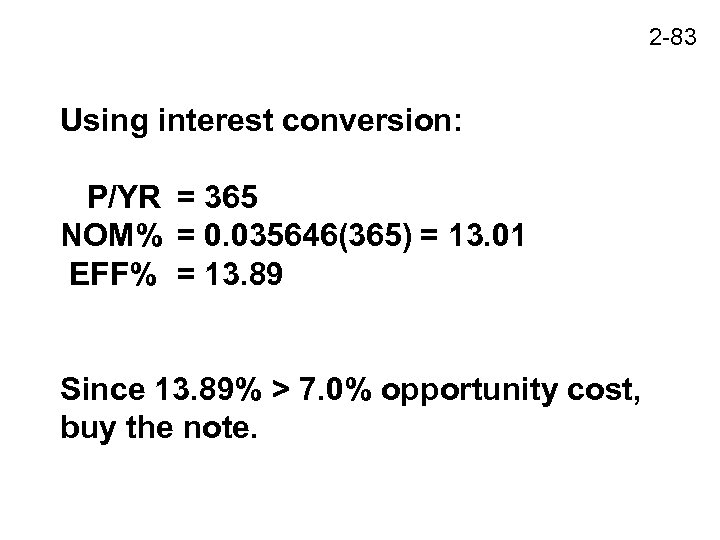2 -83 Using interest conversion: P/YR = 365 NOM% = 0. 035646(365) = 13. 01 EFF% = 13. 89 Since 13. 89% > 7. 0% opportunity cost, buy the note.